# RS Aggarwal Solutions for Class 9 Chapter 10: Quadrilaterals Exercise 10B

## RS Aggarwal Solutions for Class 9 Maths Exercise 10B PDF

RS Aggarwal solutions contain answers to all the questions which are based on the current CBSE syllabus. Each question is solved based on the evaluation process which is followed by the exam board. The students can use the RS Aggarwal Solutions to prepare for the exams more methodically. The solutions are extremely accurate and detailed in simple language to help students gain more marks. Solving the exercise wise problems and examples in RS Aggarwal textbook improve the problem solving abilities in students. RS Aggarwal Solutions for Class 9 Maths Chapter 10 Quadrilaterals Exercise 10B are provided here.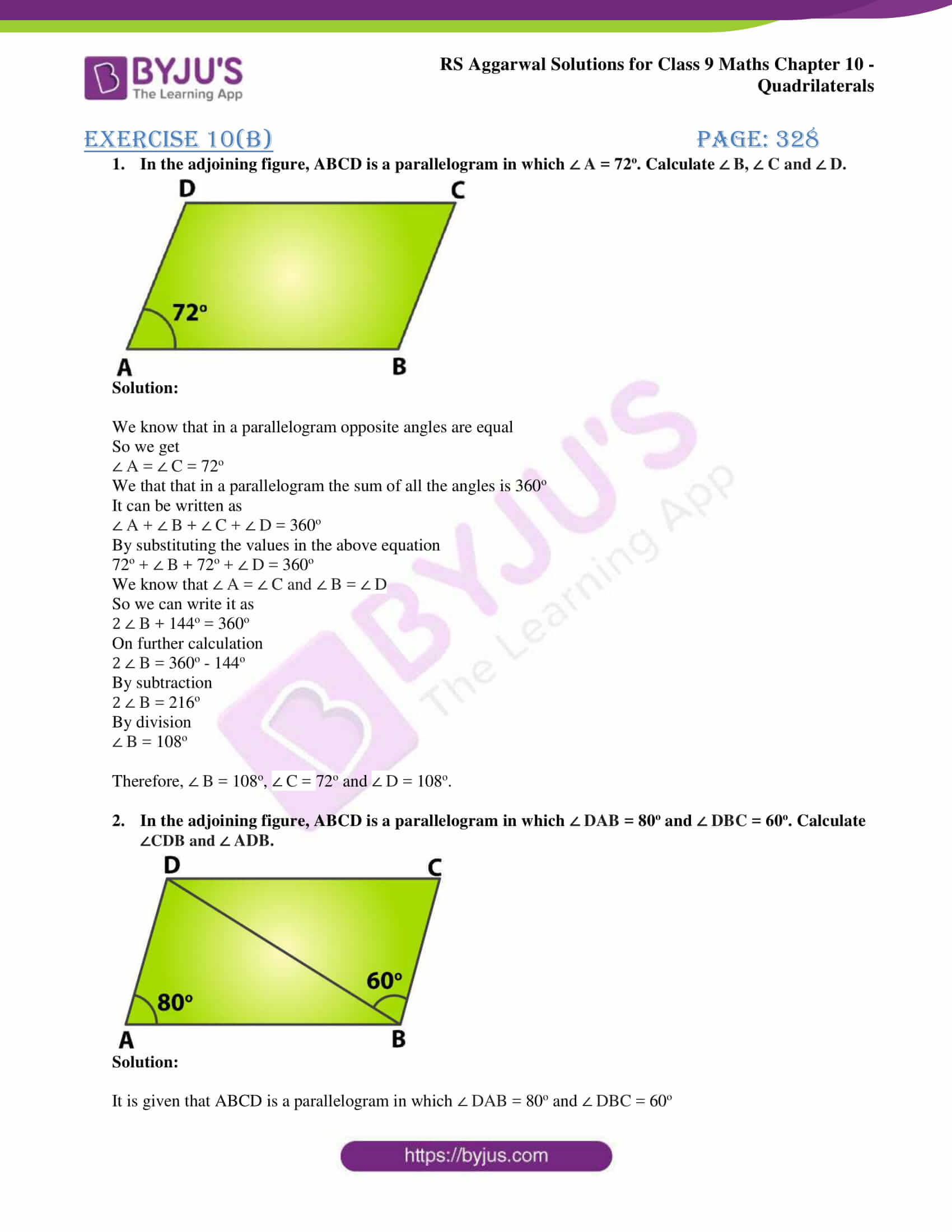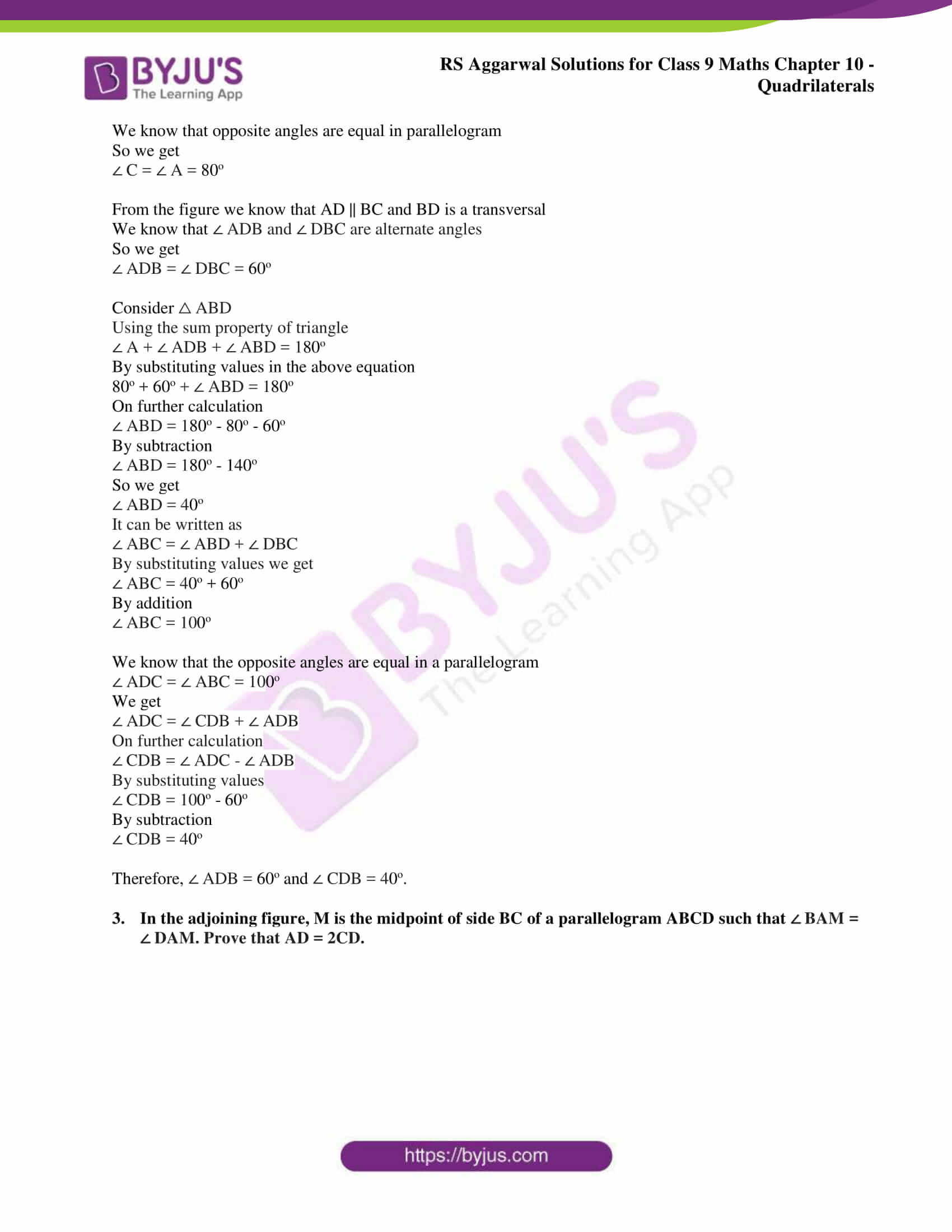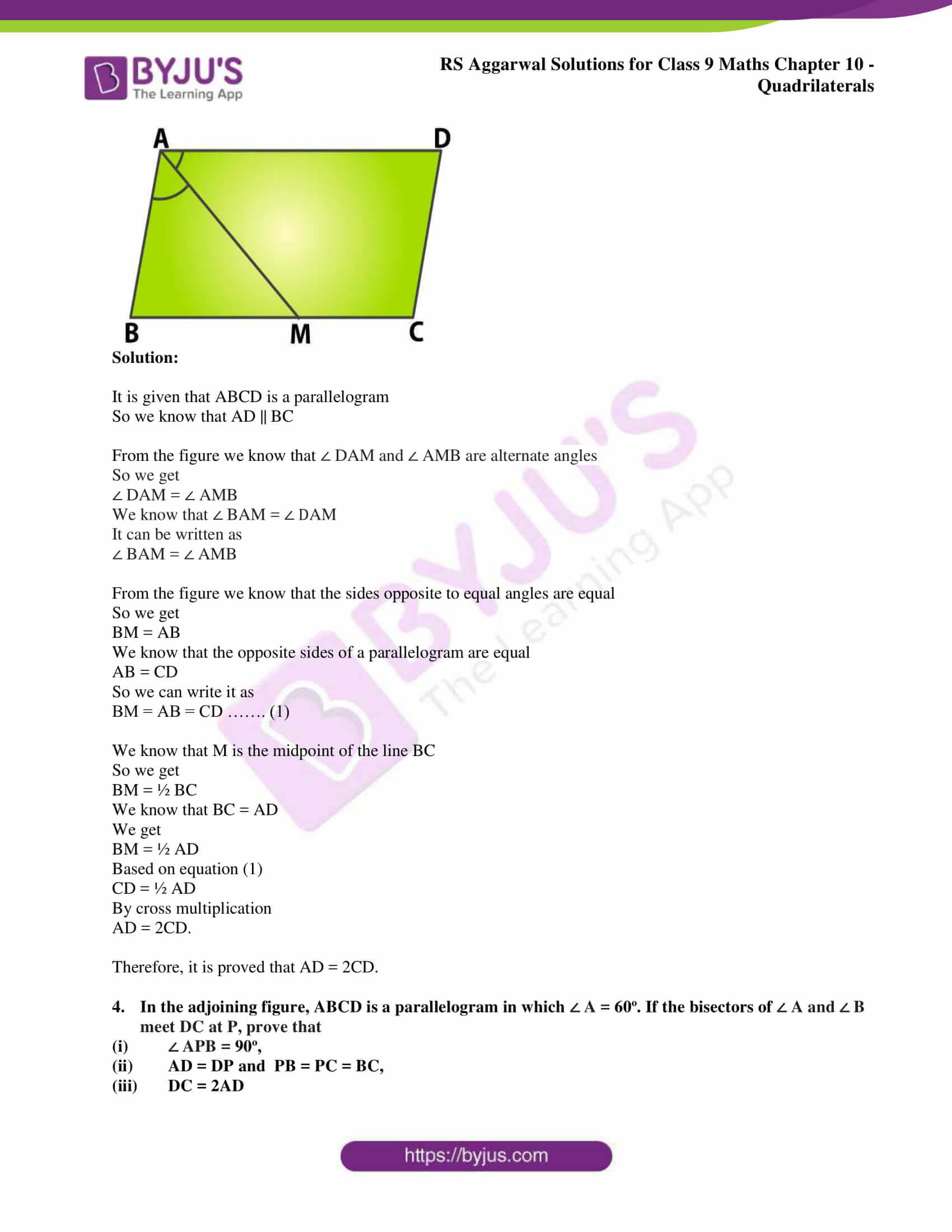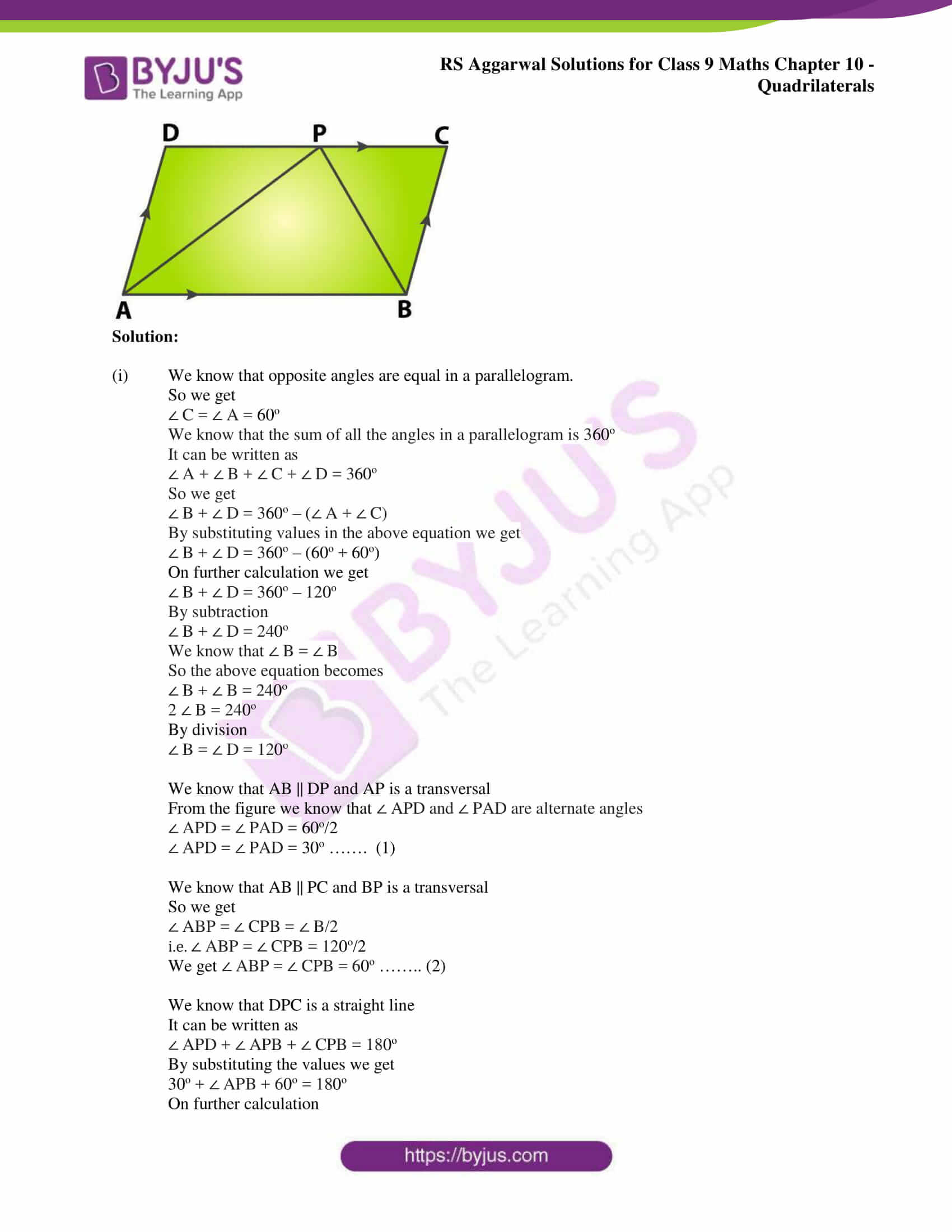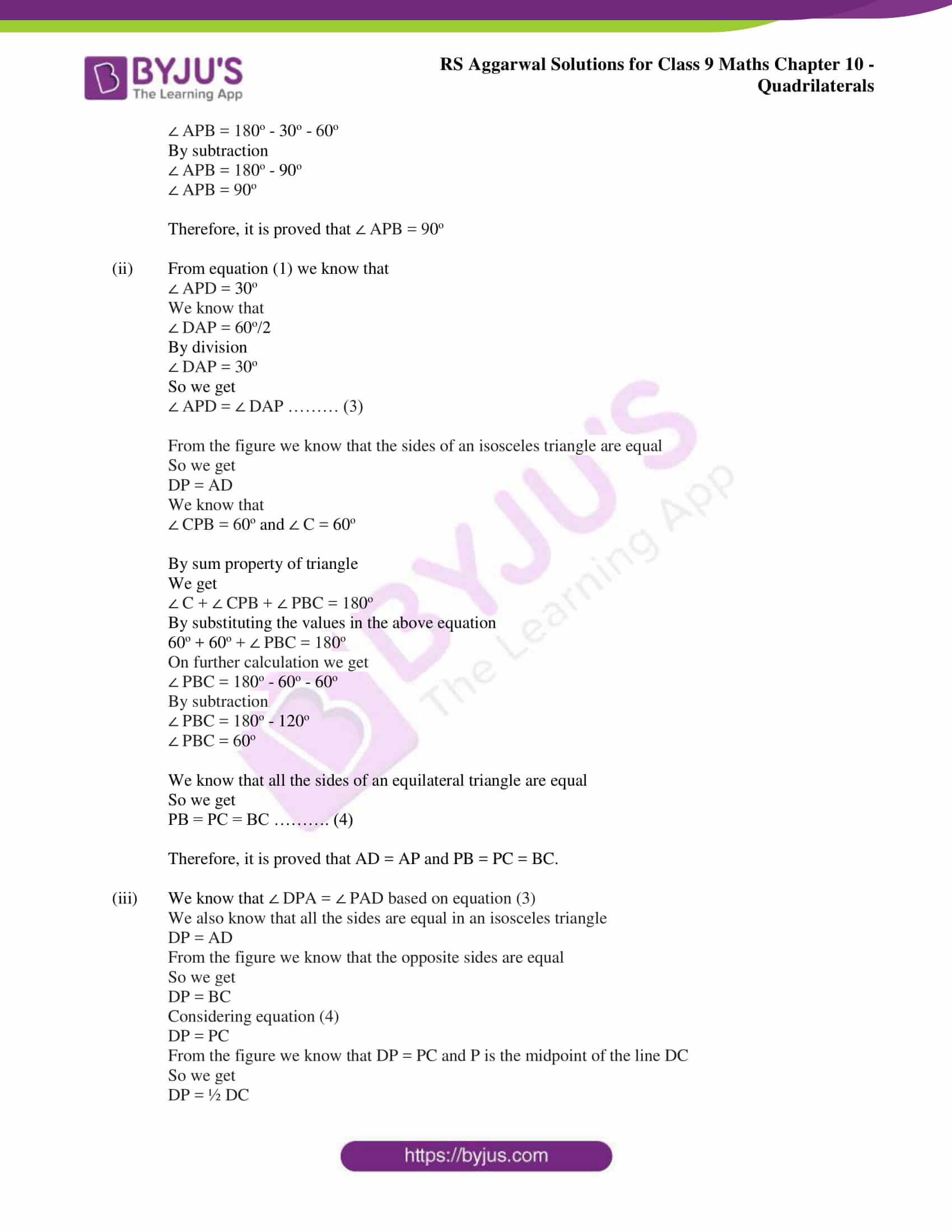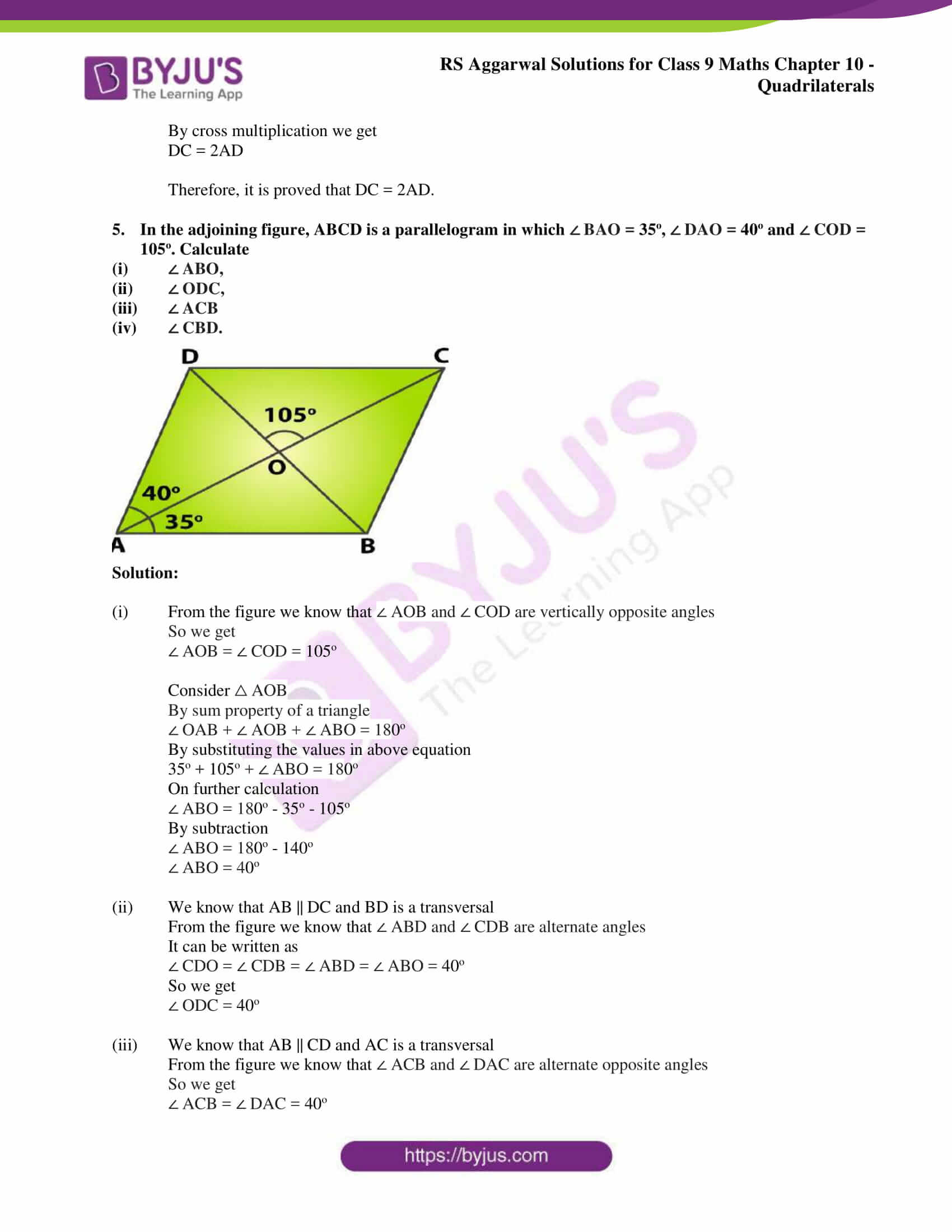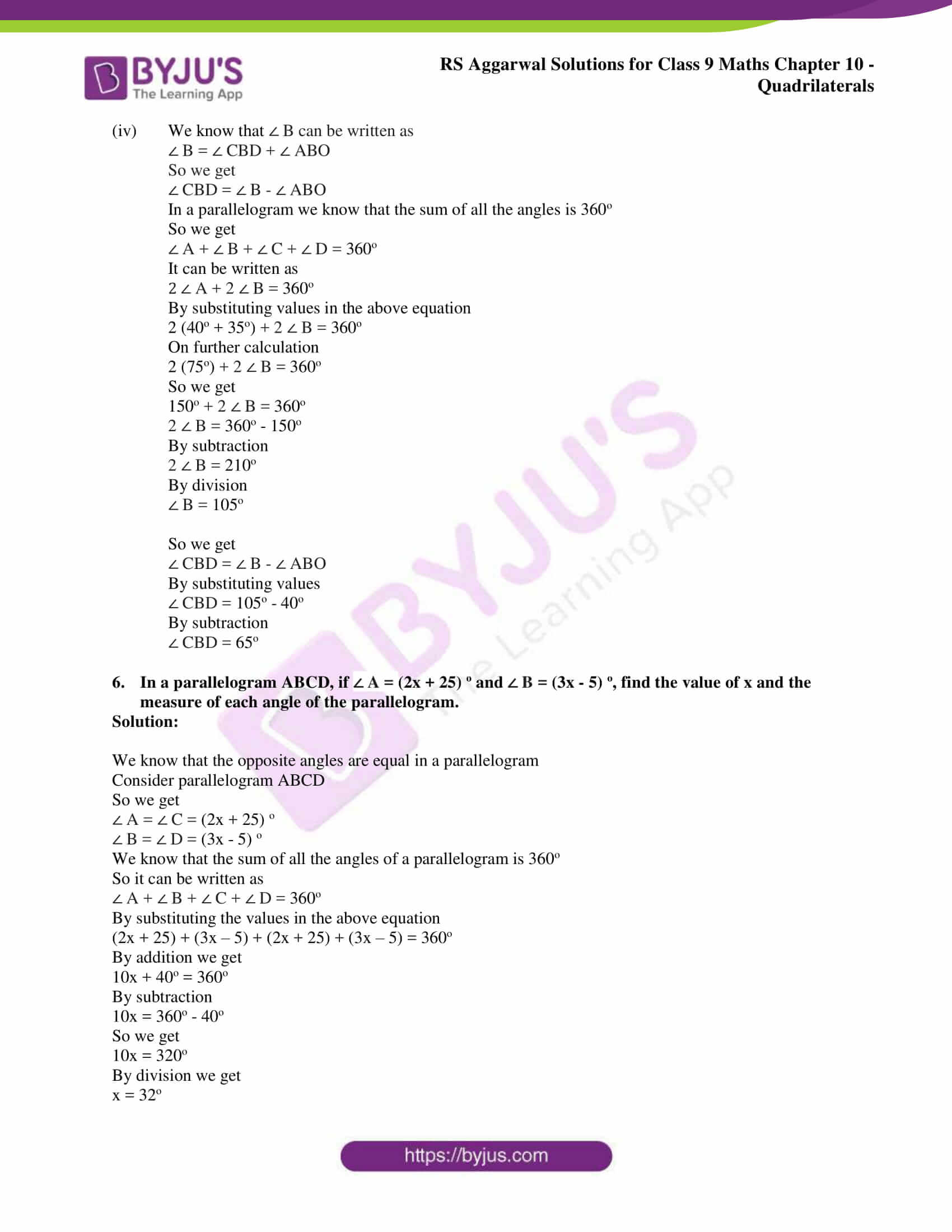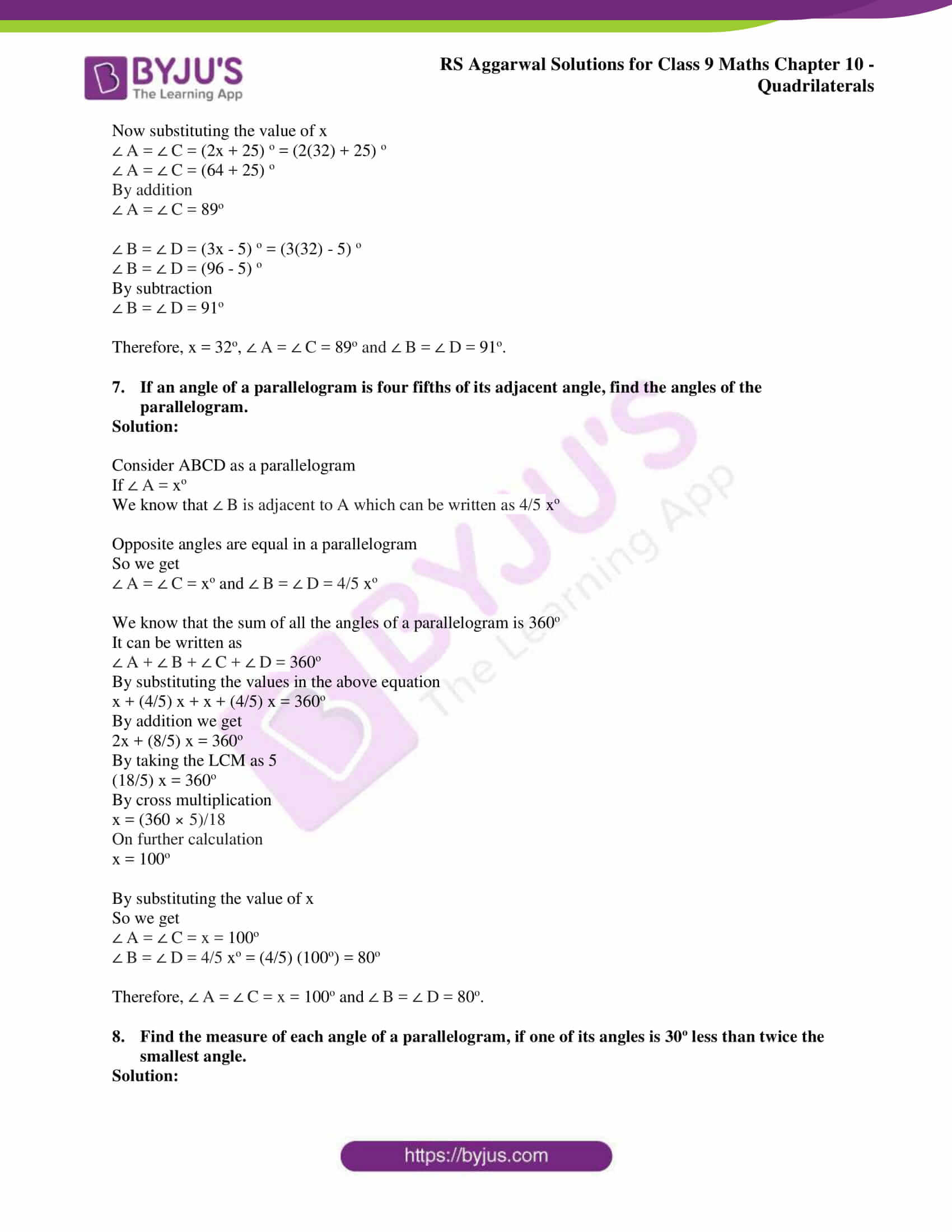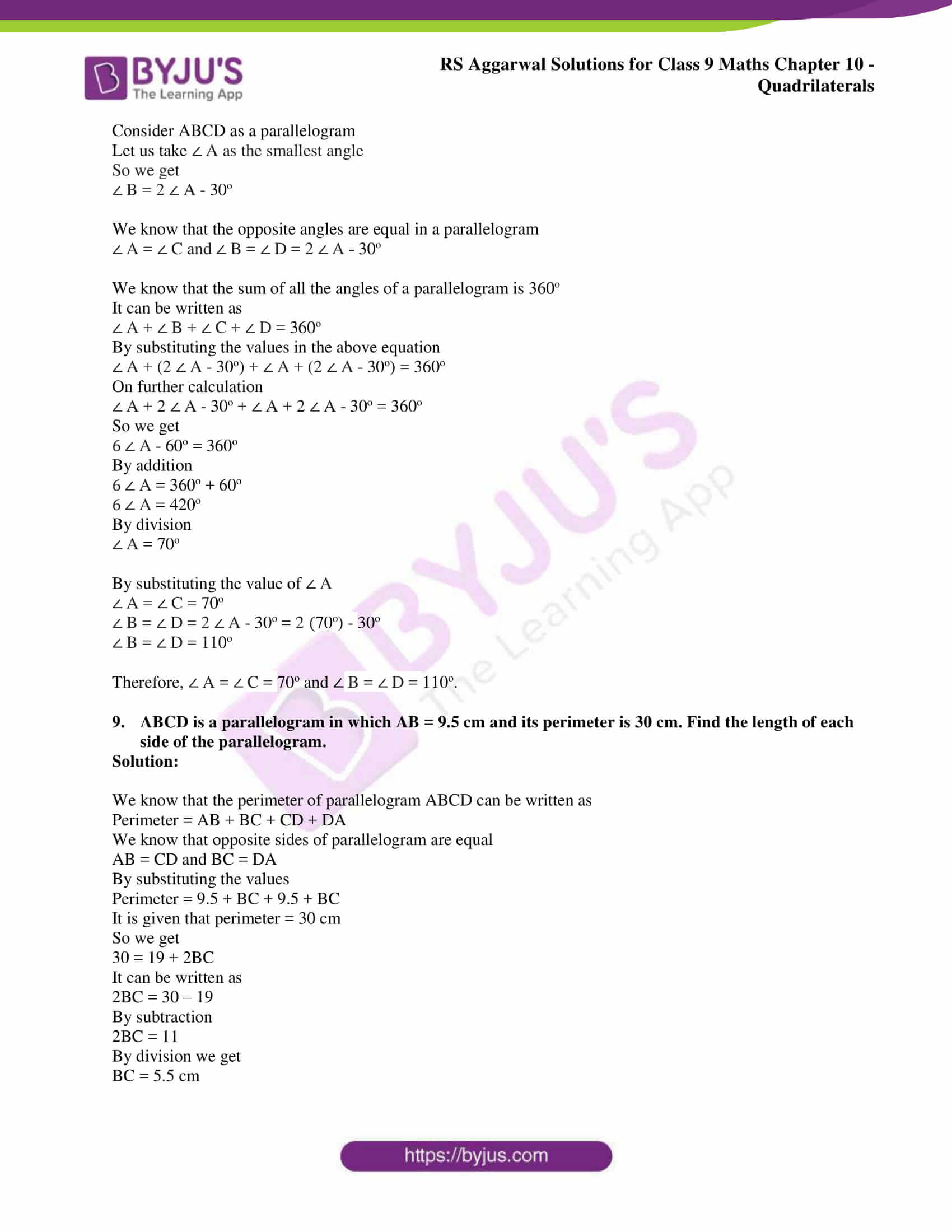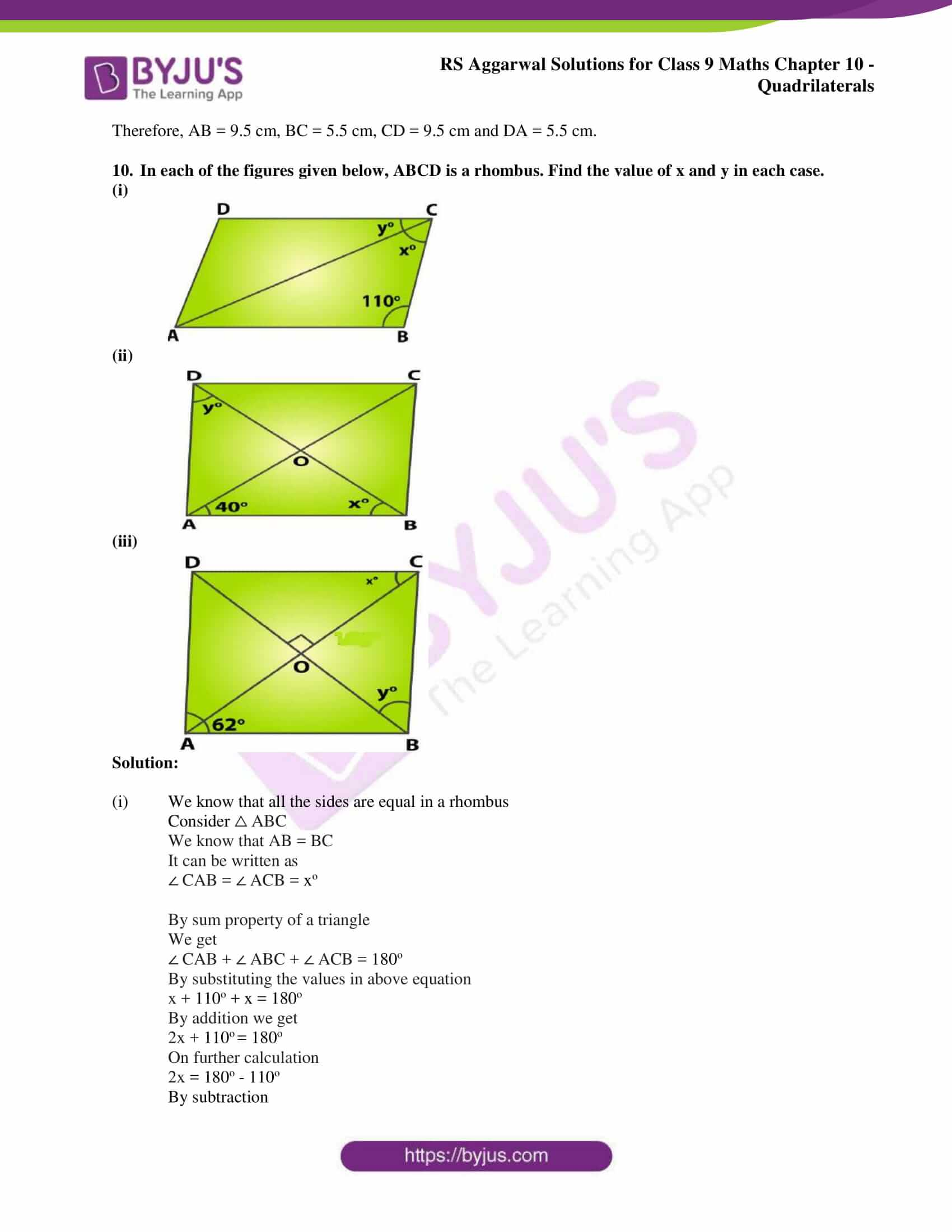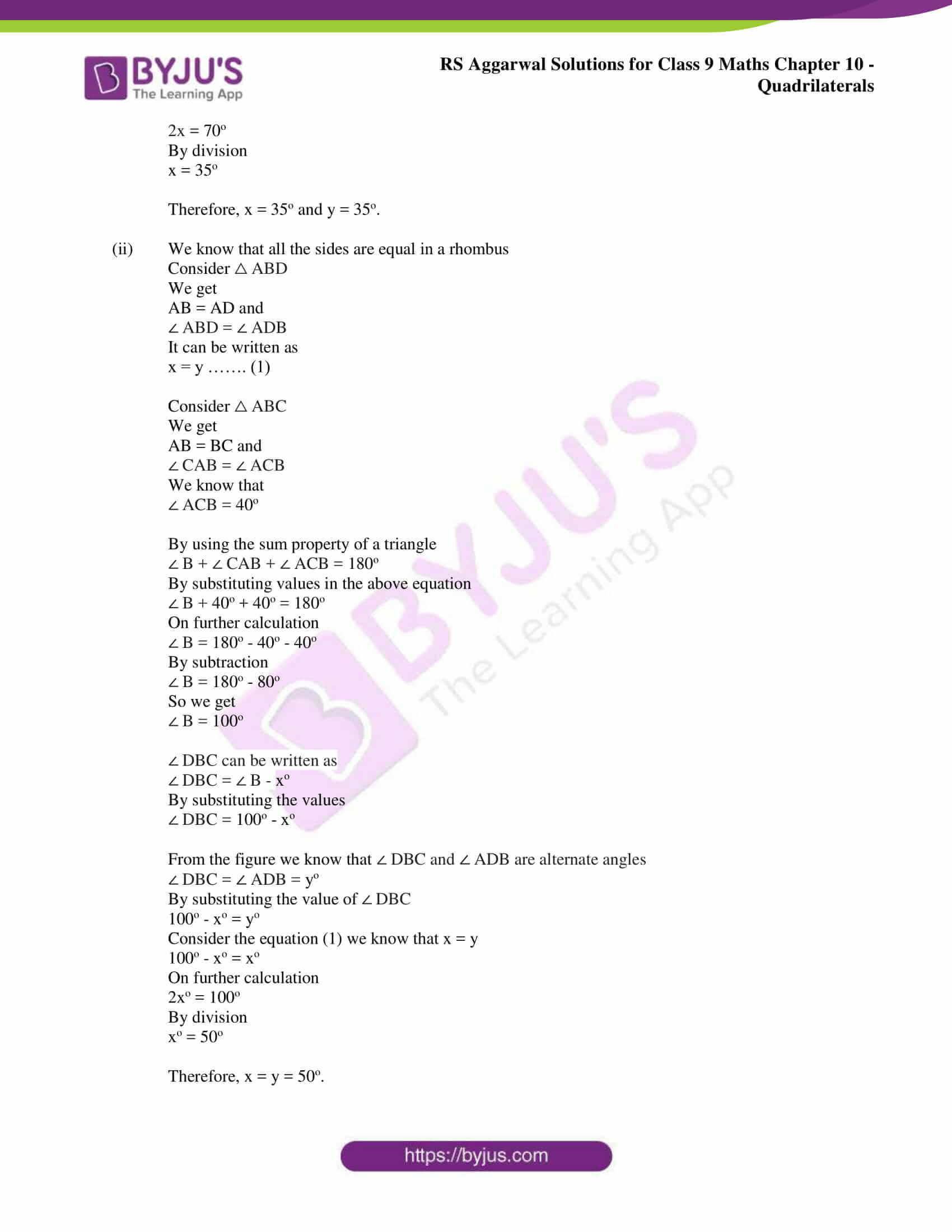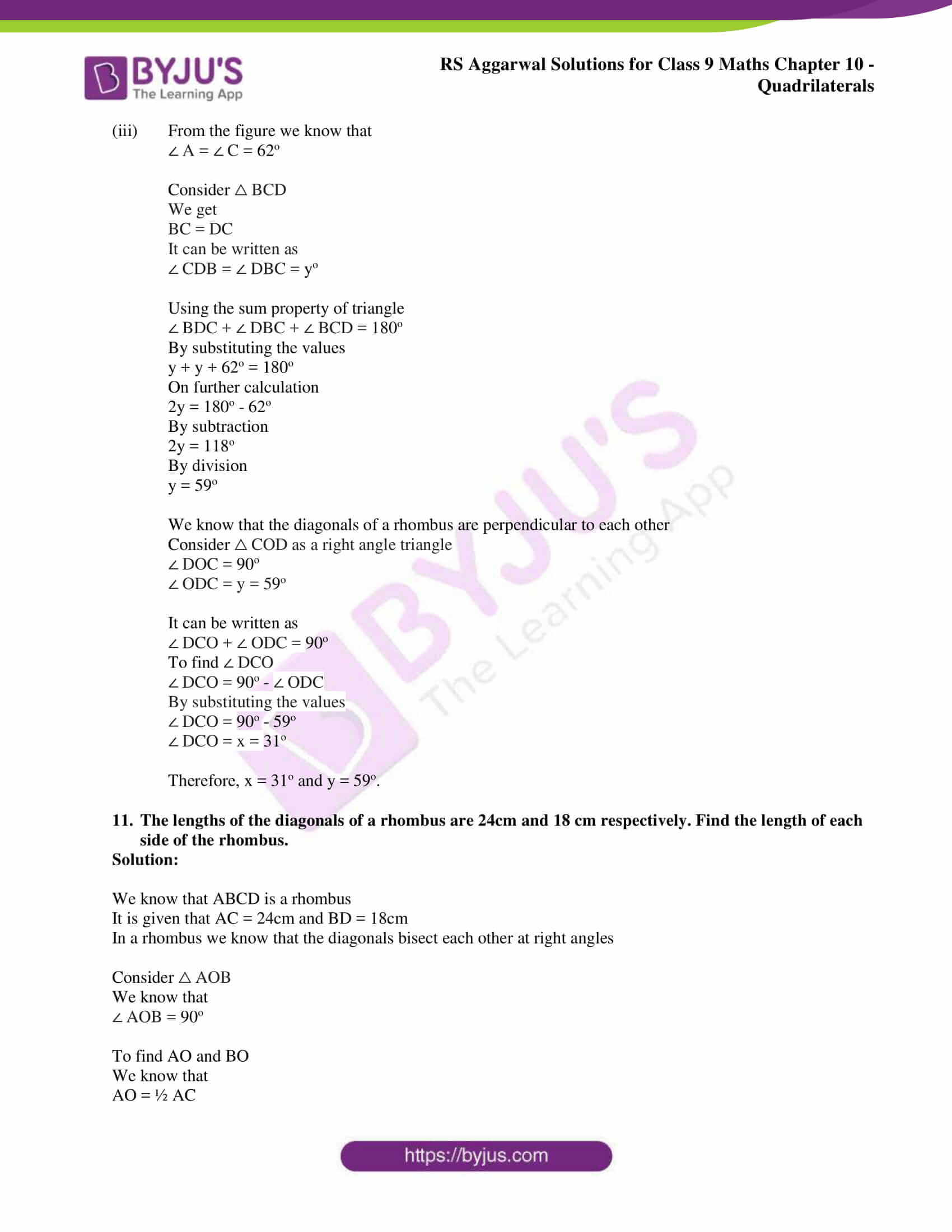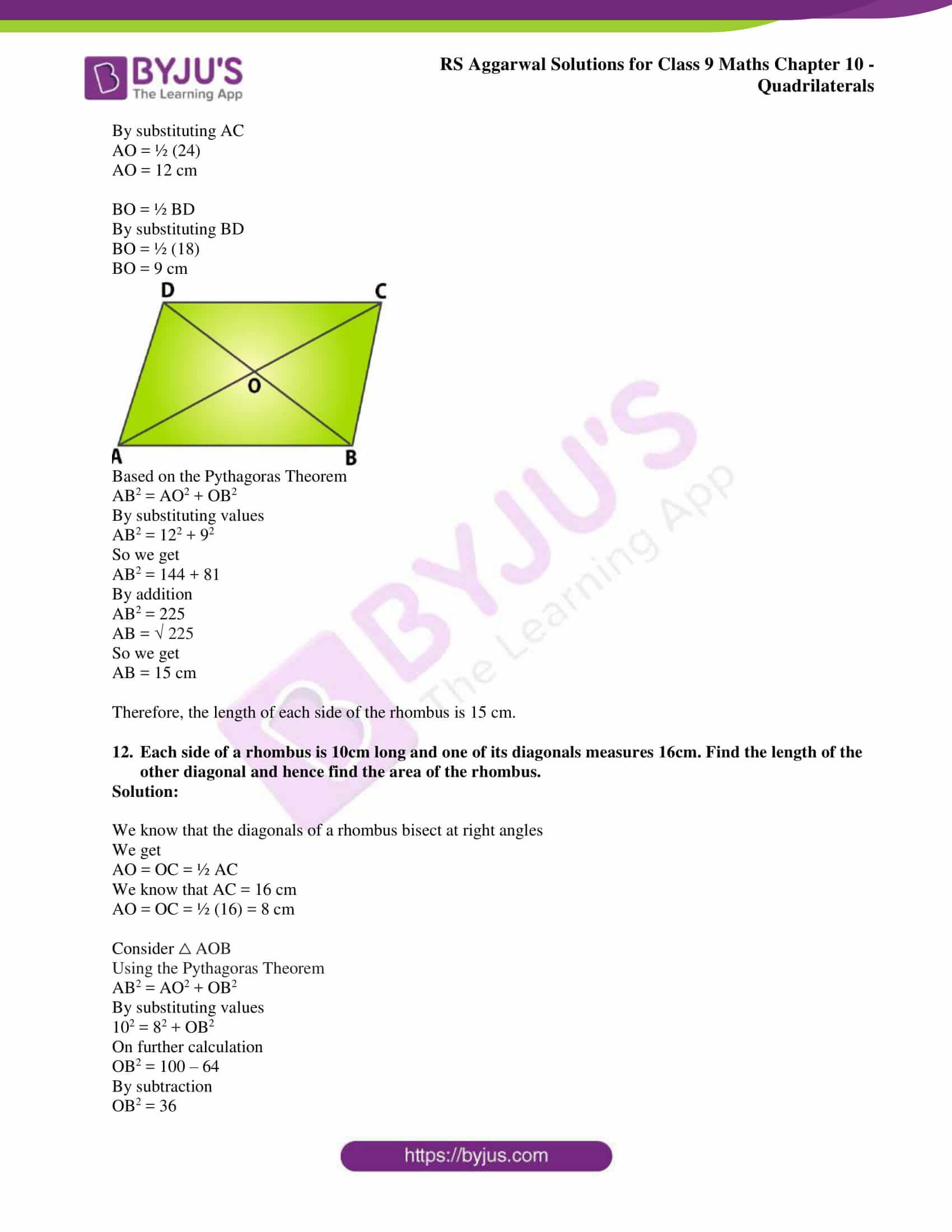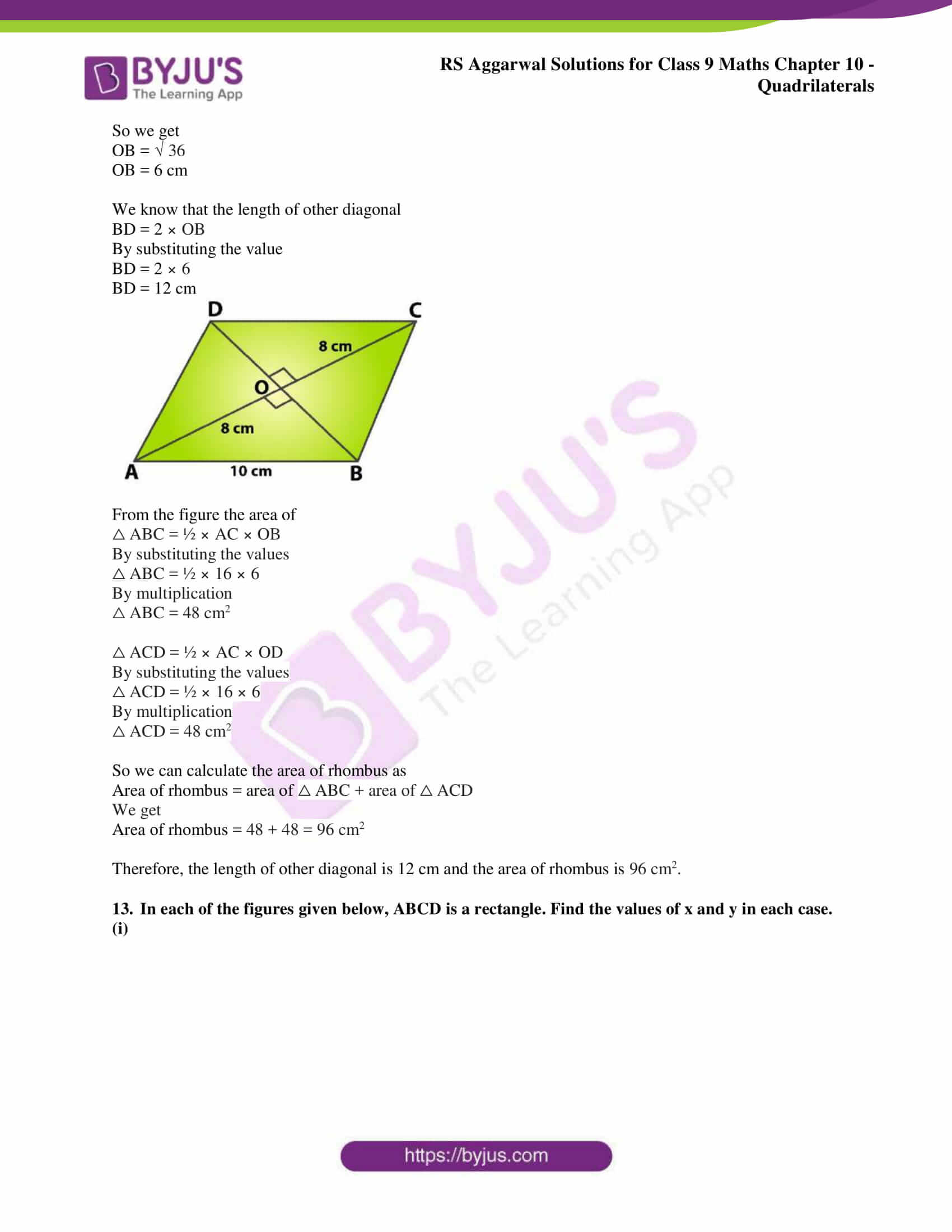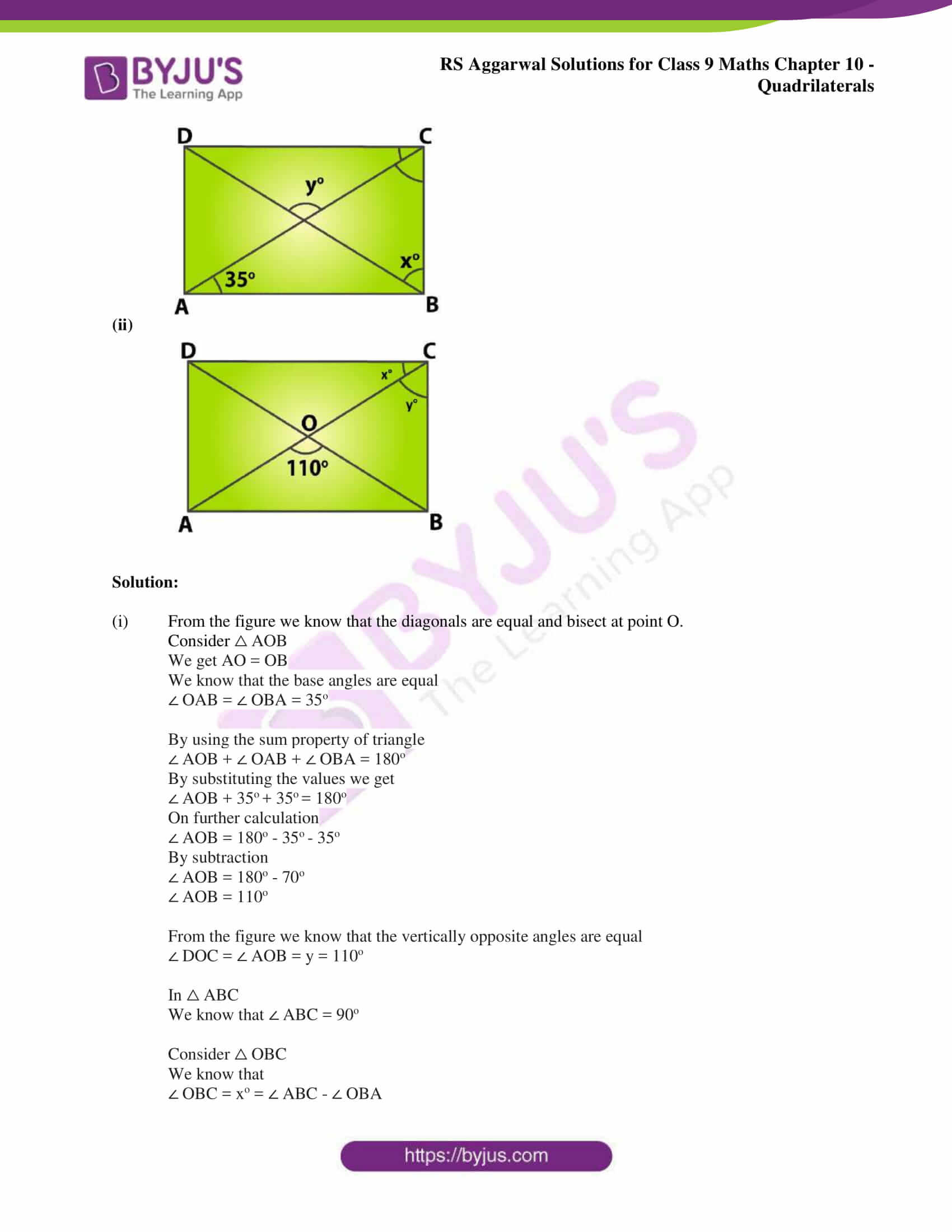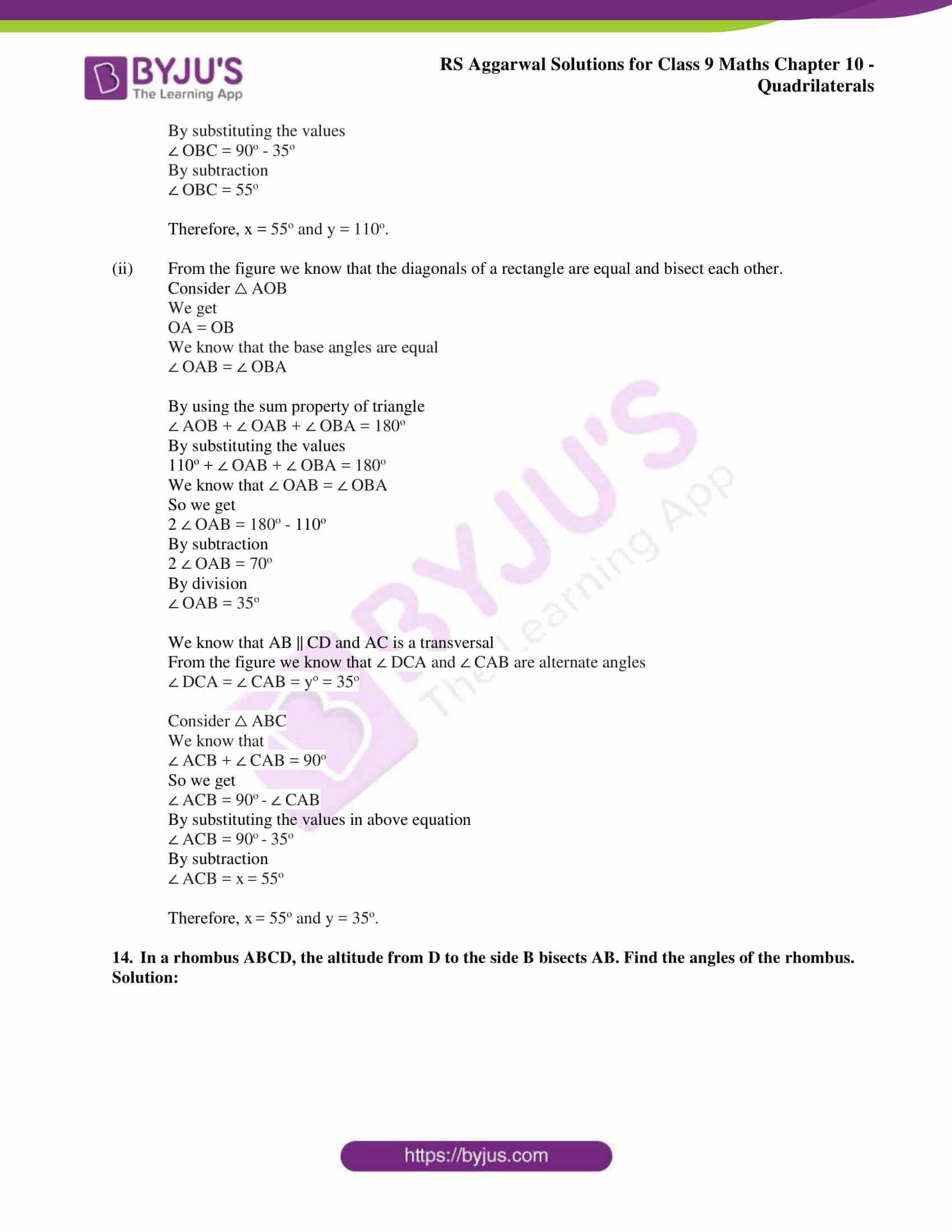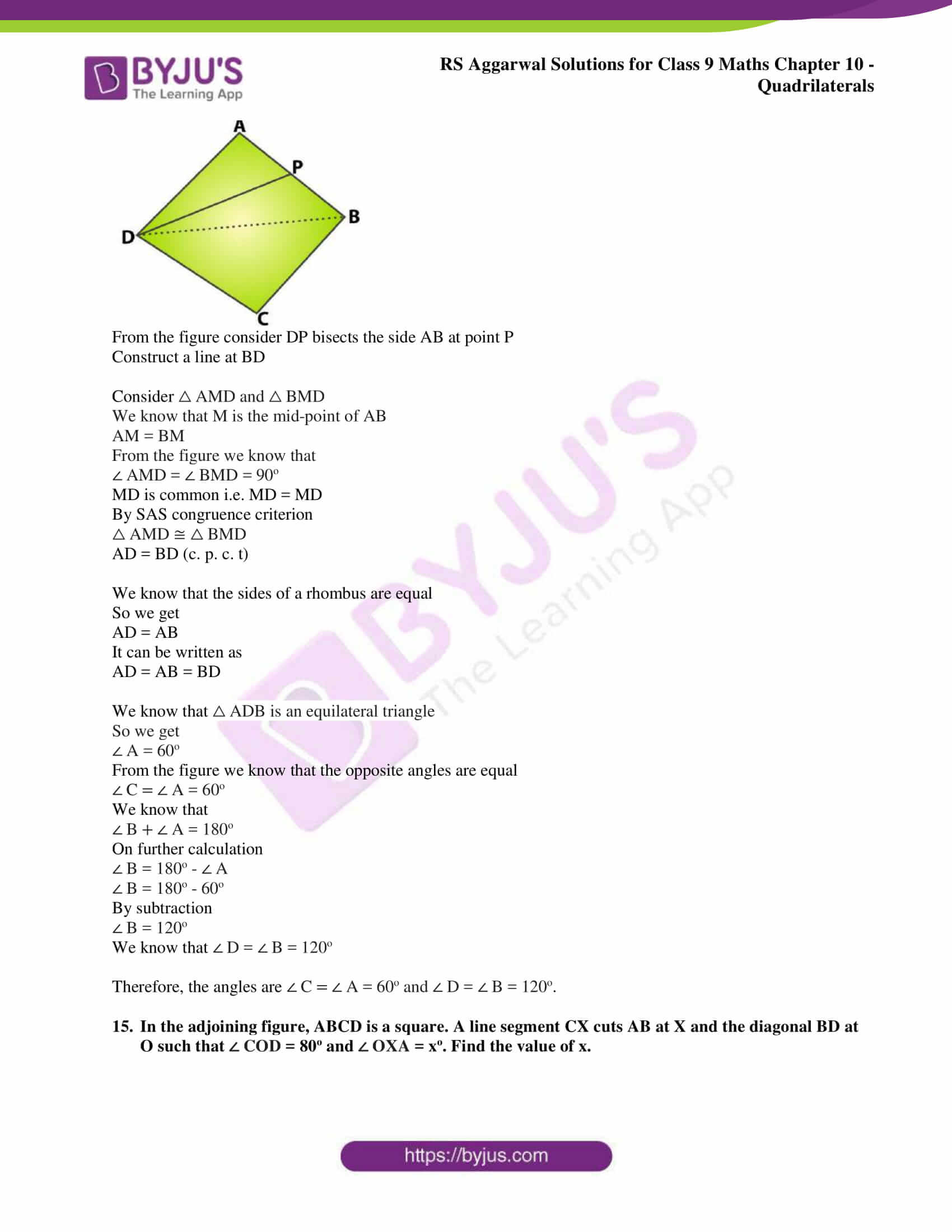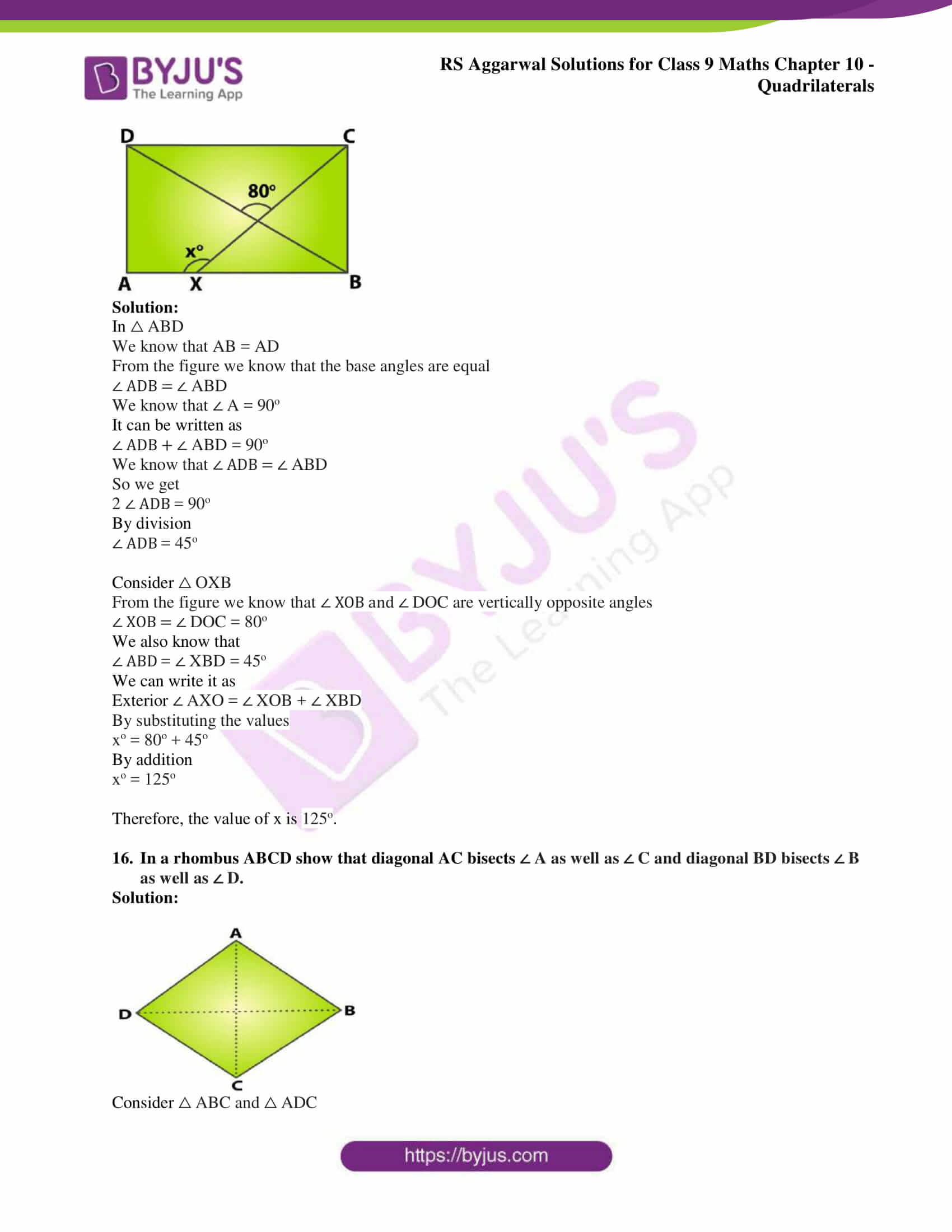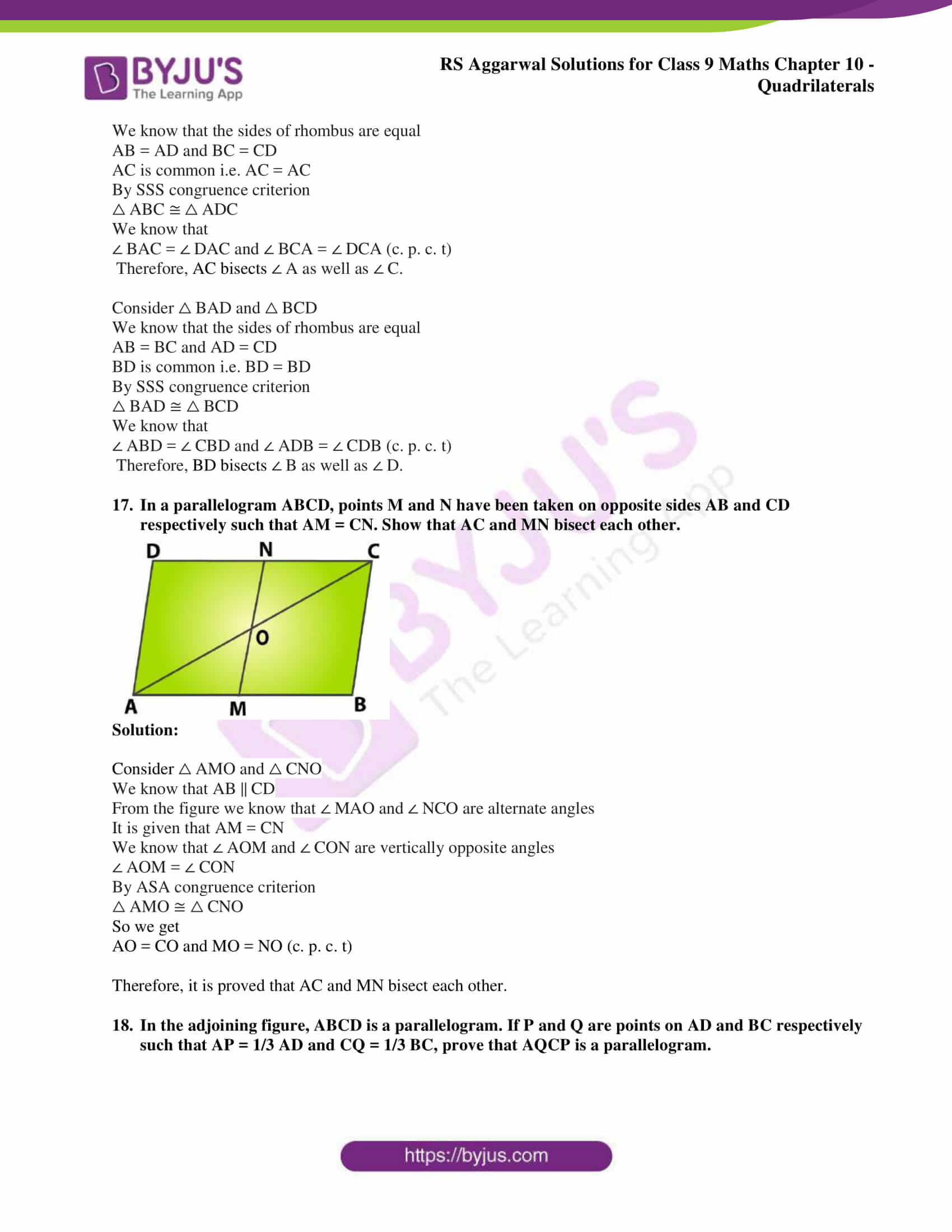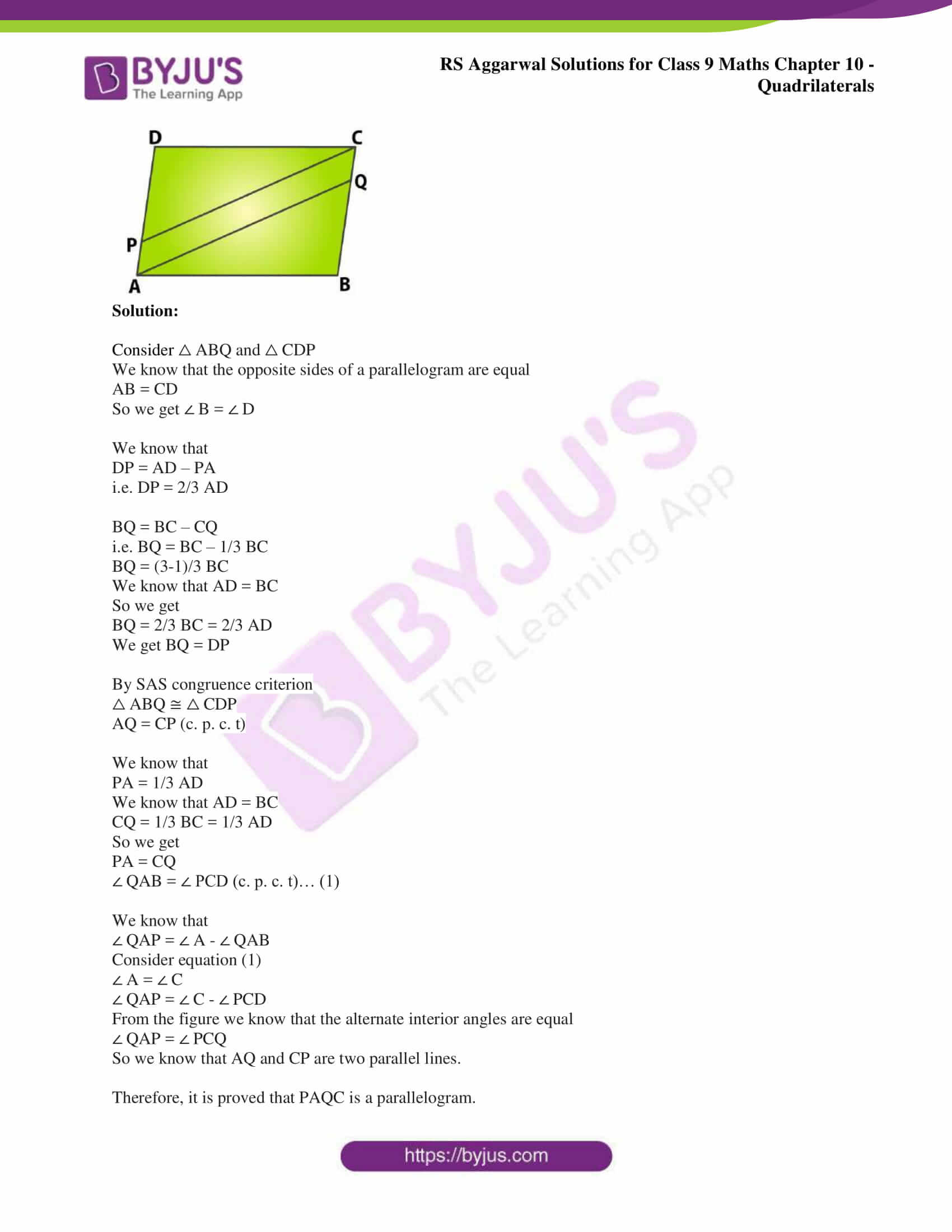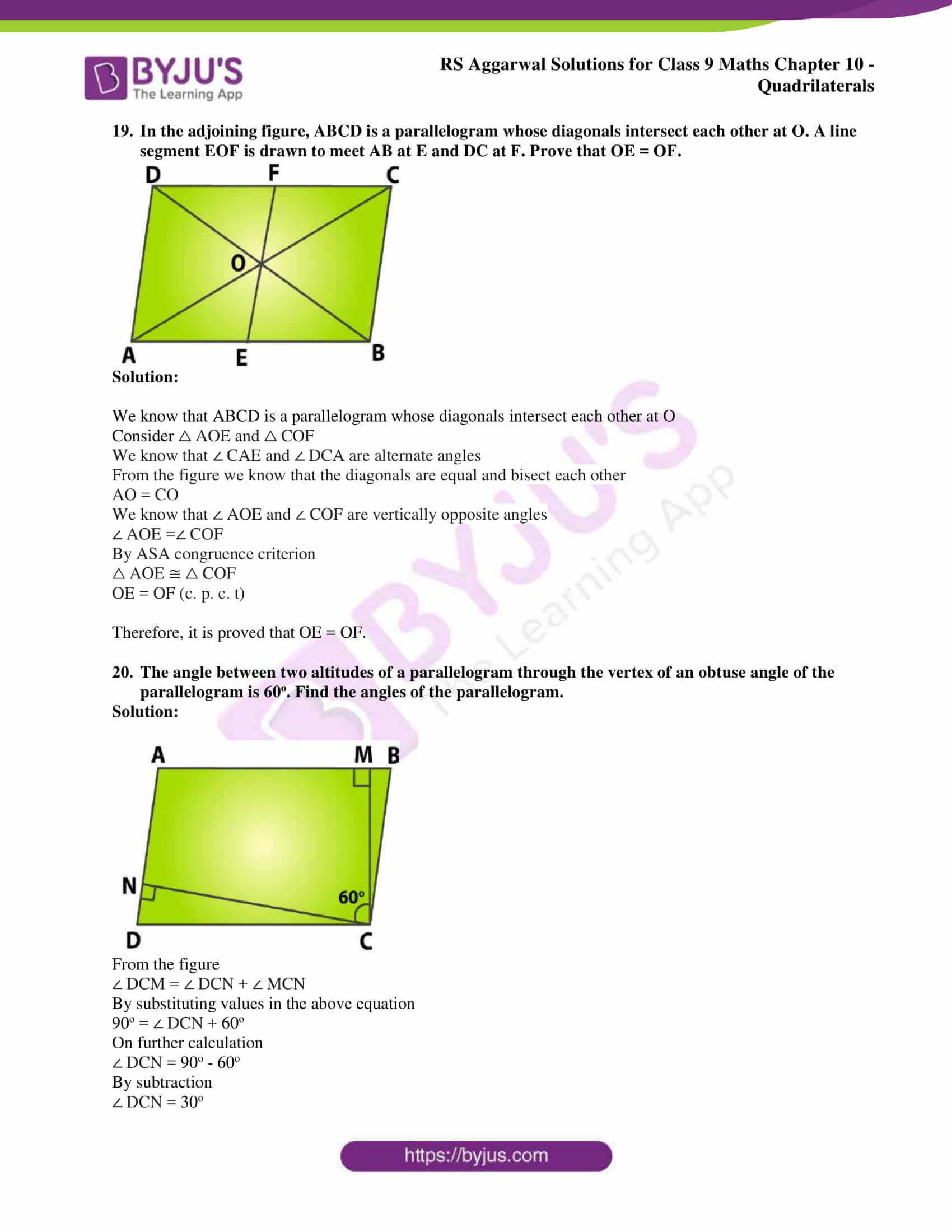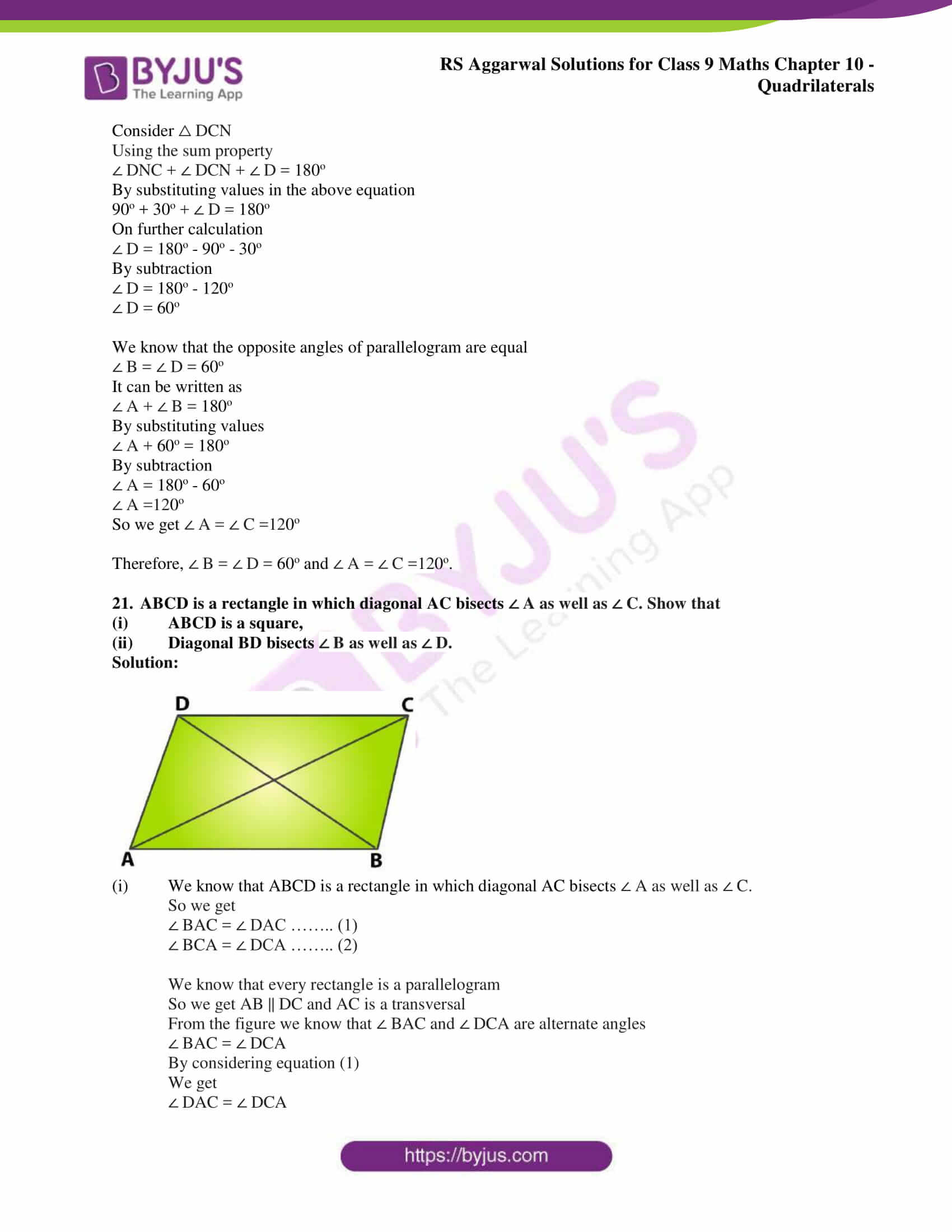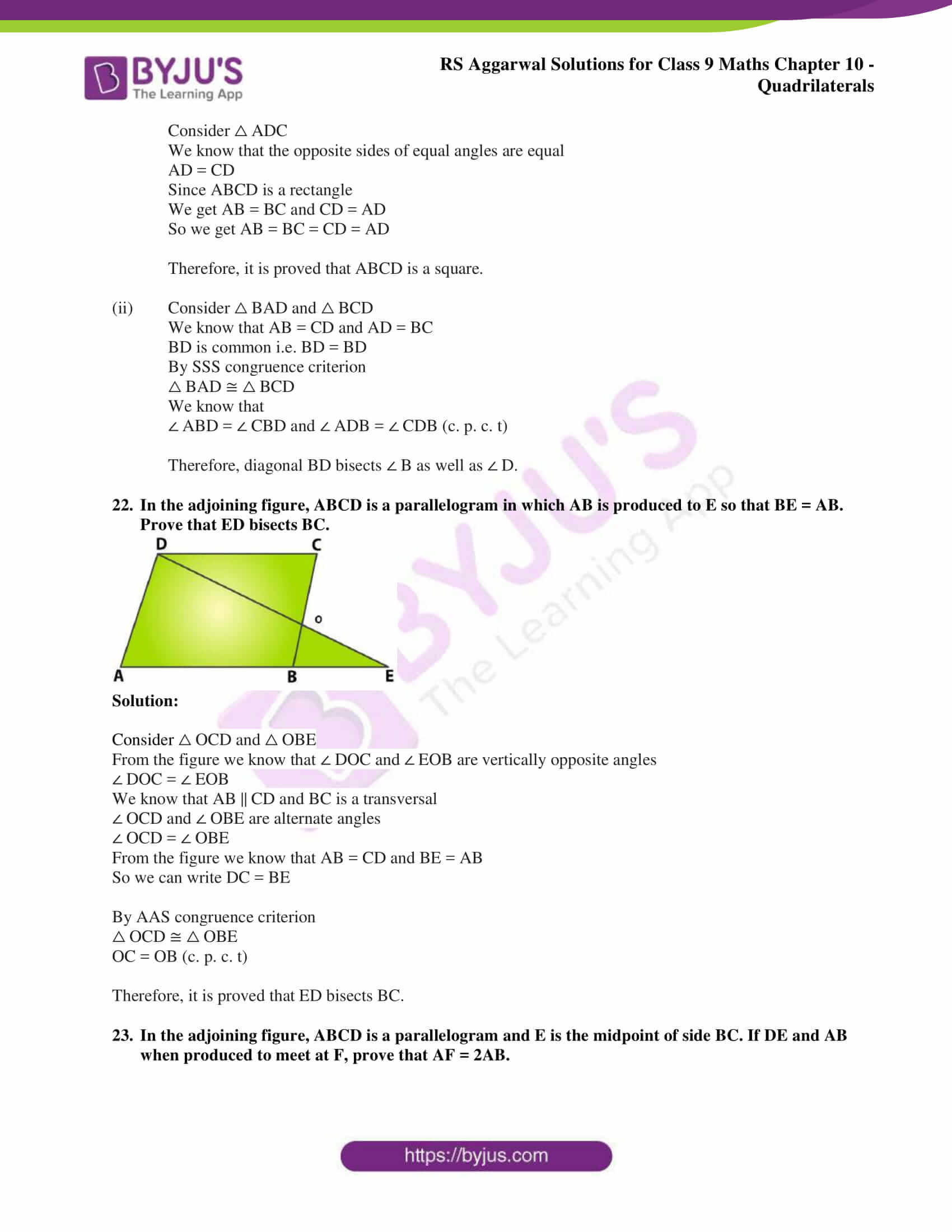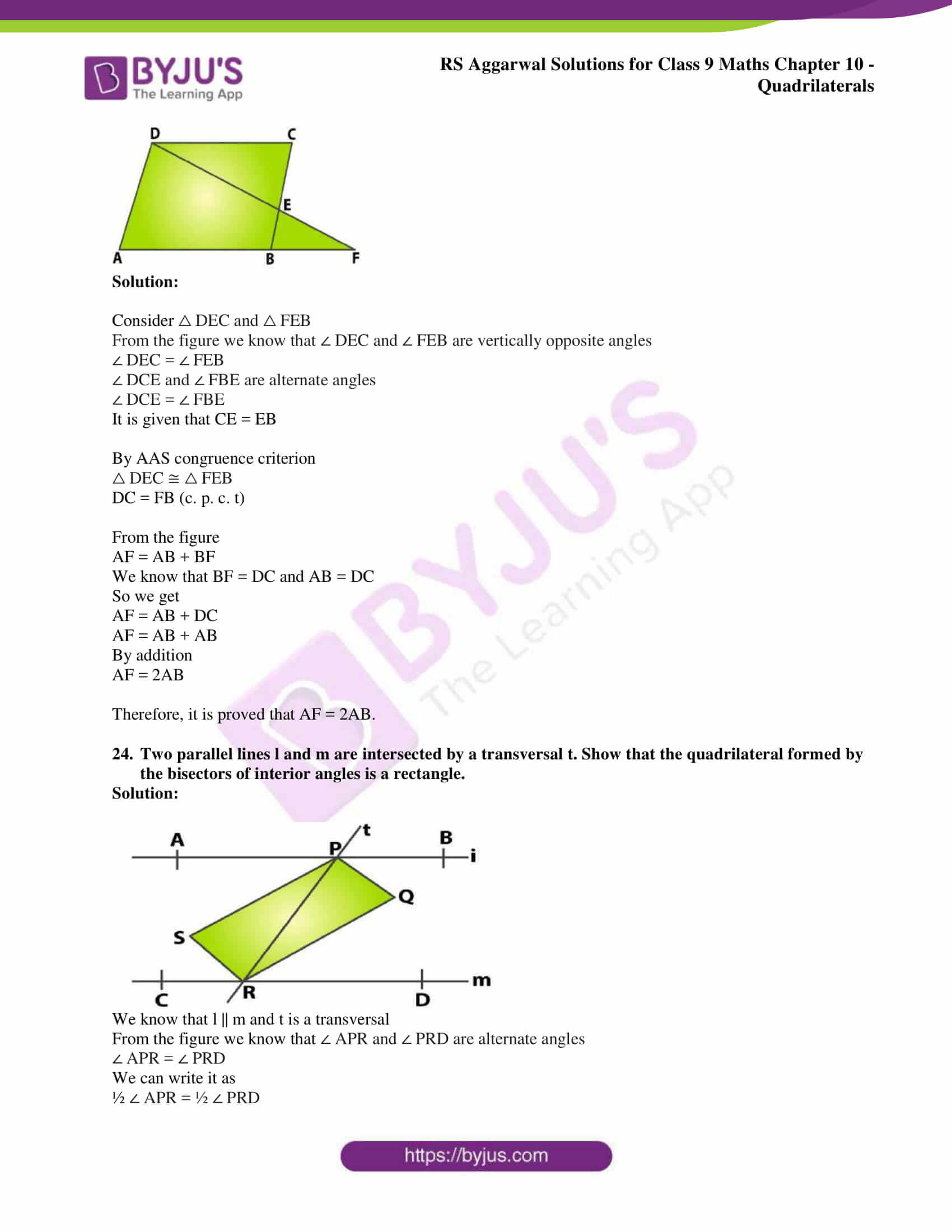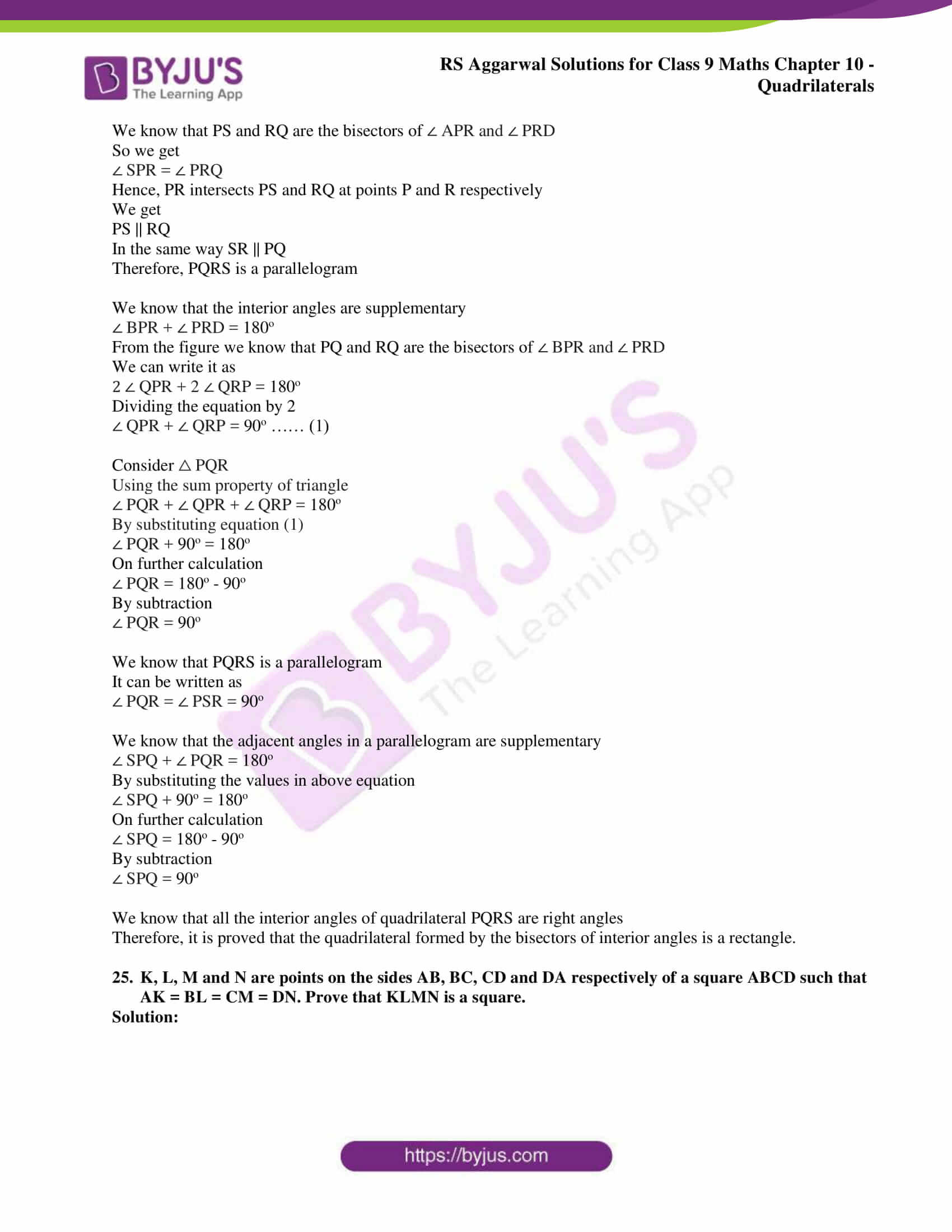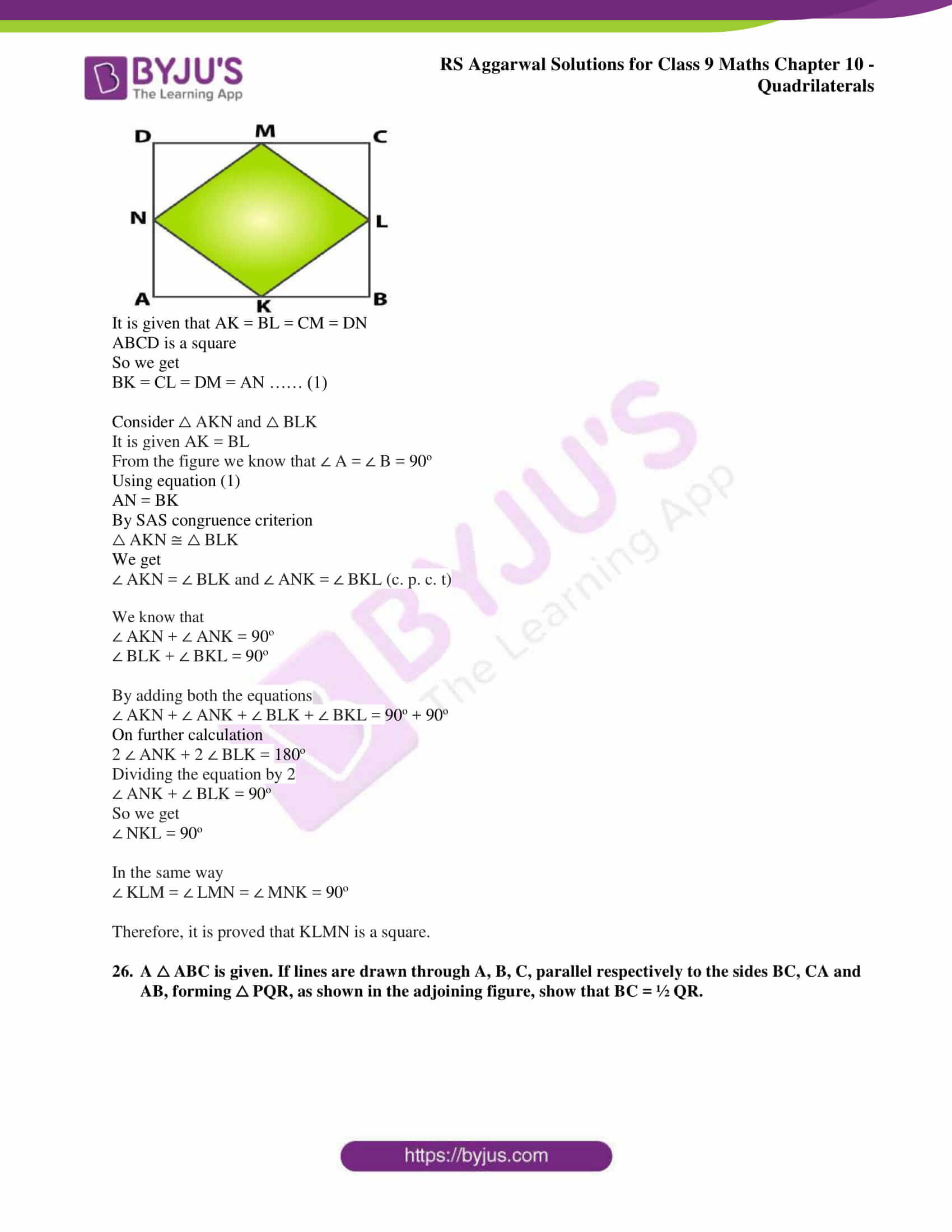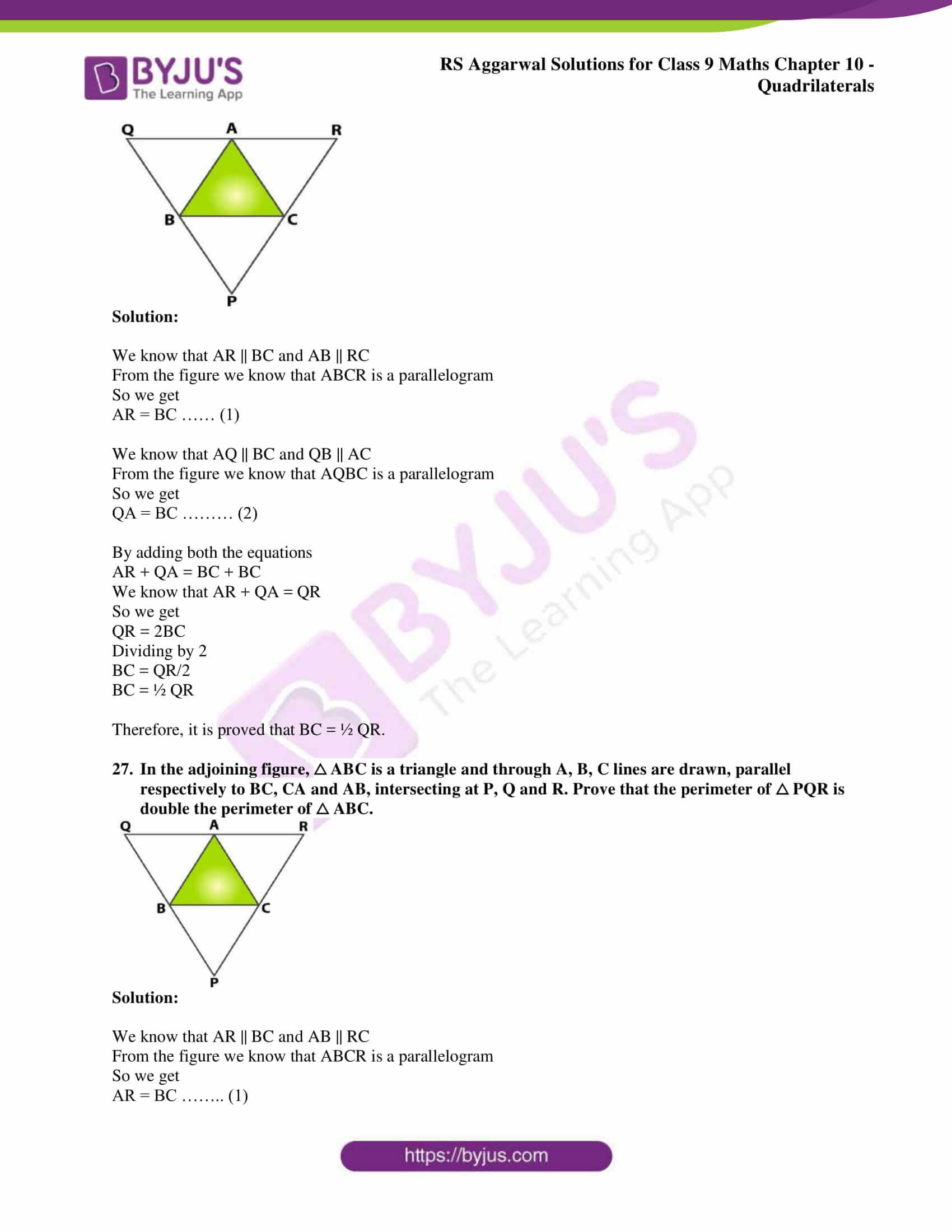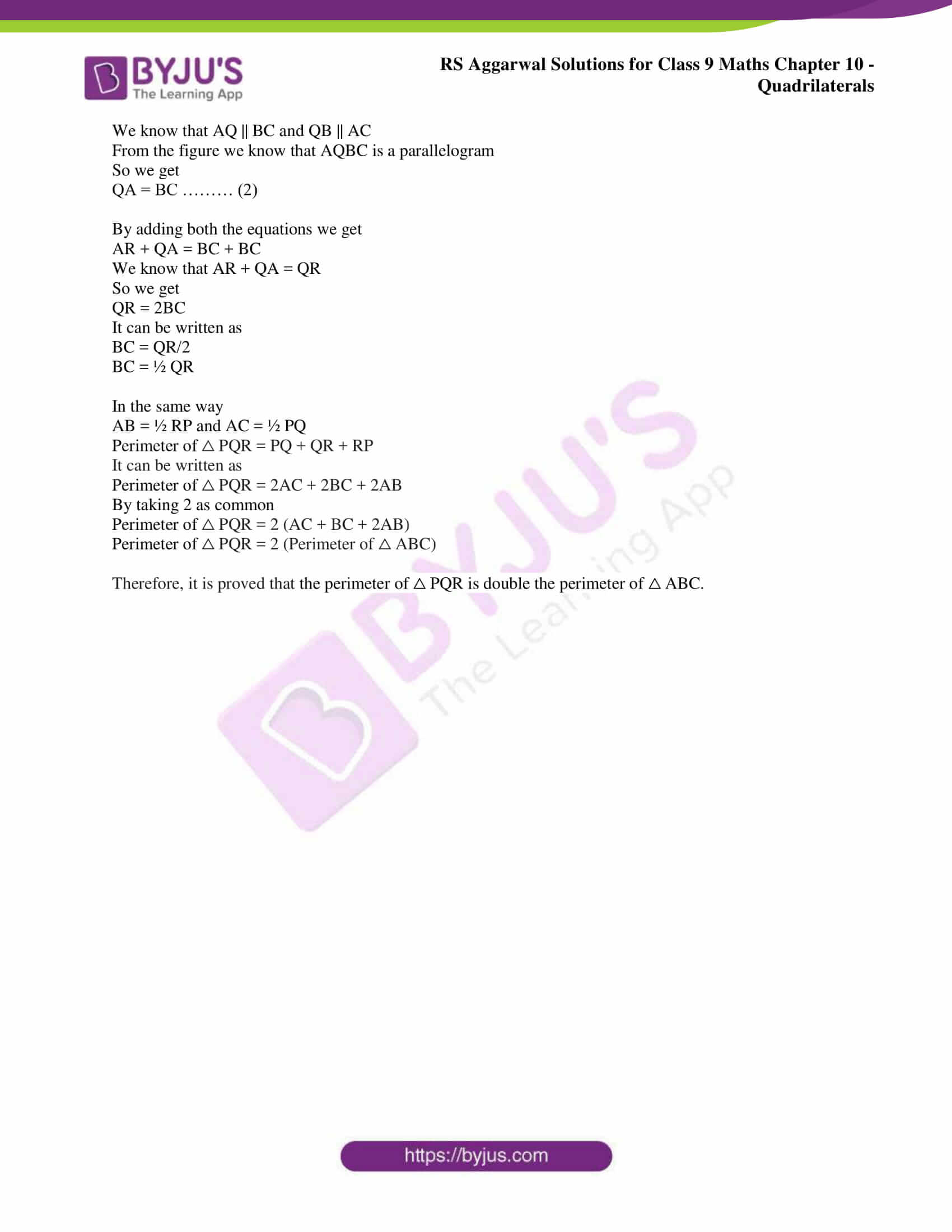## Exercise 10(B)

1. In the adjoining figure, ABCD is a parallelogram in which ∠ A = 72o. Calculate ∠ B, ∠ C and ∠ D.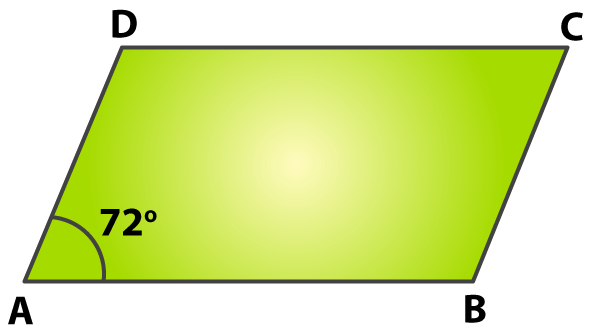Solution:

We know that in a parallelogram opposite angles are equal

So we get

∠ A = ∠ C = 72o

We that that in a parallelogram the sum of all the angles is 360o

It can be written as

∠ A + ∠ B + ∠ C + ∠ D = 360o

By substituting the values in the above equation

72o + ∠ B + 72o + ∠ D = 360o

We know that ∠ A = ∠ C and ∠ B = ∠ D

So we can write it as

2 ∠ B + 144o = 360o

On further calculation

2 ∠ B = 360o – 144o

By subtraction

2 ∠ B = 216o

By division

∠ B = 108o

Therefore, ∠ B = 108o, ∠ C = 72o and ∠ D = 108o.

2. In the adjoining figure, ABCD is a parallelogram in which ∠ DAB = 80o and ∠ DBC = 60o. Calculate ∠CDB and ∠ ADB.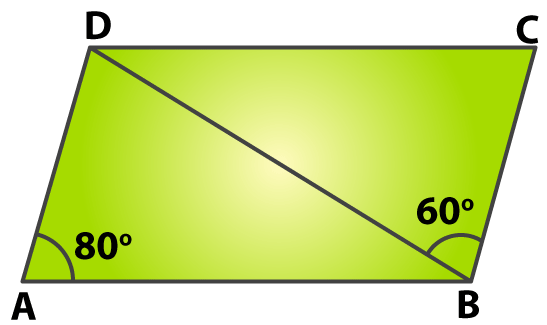Solution:

It is given that ABCD is a parallelogram in which ∠ DAB = 80o and ∠ DBC = 60o

We know that opposite angles are equal in parallelogram

So we get

∠ C = ∠ A = 80o

From the figure we know that AD || BC and BD is a transversal

We know that ∠ ADB and ∠ DBC are alternate angles

So we get

∠ ADB = ∠ DBC = 60o

Consider △ ABD

Using the sum property of triangle

∠ A + ∠ ADB + ∠ ABD = 180o

By substituting values in the above equation

80o + 60o + ∠ ABD = 180o

On further calculation

∠ ABD = 180o – 80o – 60o

By subtraction

∠ ABD = 180o – 140o

So we get

∠ ABD = 40o

It can be written as

∠ ABC = ∠ ABD + ∠ DBC

By substituting values we get

∠ ABC = 40o + 60o

∠ ABC = 100o

We know that the opposite angles are equal in a parallelogram

∠ ADC = ∠ ABC = 100o

We get

On further calculation

By substituting values

∠ CDB = 100o – 60o

By subtraction

∠ CDB = 40o

Therefore, ∠ ADB = 60o and ∠ CDB = 40o.

3. In the adjoining figure, M is the midpoint of side BC of a parallelogram ABCD such that ∠ BAM = ∠ DAM. Prove that AD = 2CD.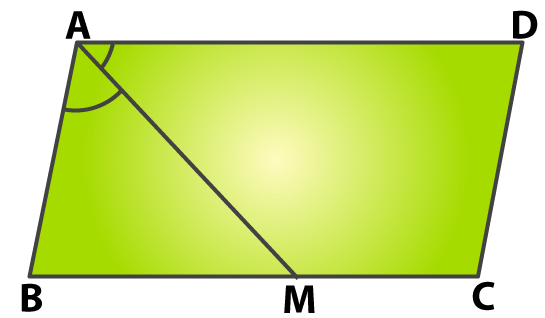Solution:

It is given that ABCD is a parallelogram

So we know that AD || BC

From the figure we know that ∠ DAM and ∠ AMB are alternate angles

So we get

∠ DAM = ∠ AMB

We know that ∠ BAM = ∠ DAM

It can be written as

∠ BAM = ∠ AMB

From the figure we know that the sides opposite to equal angles are equal

So we get

BM = AB

We know that the opposite sides of a parallelogram are equal

AB = CD

So we can write it as

BM = AB = CD ……. (1)

We know that M is the midpoint of the line BC

So we get

BM = ½ BC

We know that BC = AD

We get

Based on equation (1)

By cross multiplication

Therefore, it is proved that AD = 2CD.

4. In the adjoining figure, ABCD is a parallelogram in which ∠ A = 60o. If the bisectors of ∠ A and ∠ B meet DC at P, prove that

(i) ∠ APB = 90o,

(ii) AD = DP and PB = PC = BC,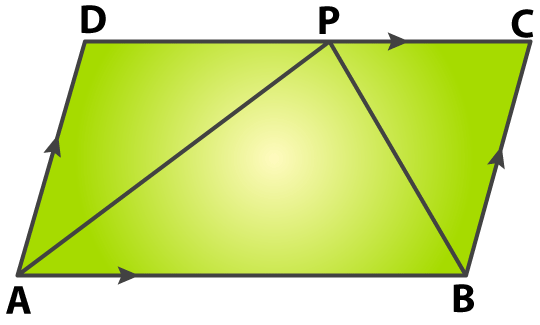Solution:

(i) We know that opposite angles are equal in a parallelogram.

So we get

∠ C = ∠ A = 60o

We know that the sum of all the angles in a parallelogram is 360o

It can be written as

∠ A + ∠ B + ∠ C + ∠ D = 360o

So we get

∠ B + ∠ D = 360o – (∠ A + ∠ C)

By substituting values in the above equation we get

∠ B + ∠ D = 360o – (60o + 60o)

On further calculation we get

∠ B + ∠ D = 360o – 120o

By subtraction

∠ B + ∠ D = 240o

We know that ∠ B = ∠ B

So the above equation becomes

∠ B + ∠ B = 240o

2 ∠ B = 240o

By division

∠ B = ∠ D = 120o

We know that AB || DP and AP is a transversal

From the figure we know that ∠ APD and ∠ PAD are alternate angles

∠ APD = ∠ PAD = 60o/2

∠ APD = ∠ PAD = 30o ……. (1)

We know that AB || PC and BP is a transversal

So we get

∠ ABP = ∠ CPB = ∠ B/2

i.e. ∠ ABP = ∠ CPB = 120o/2

We get ∠ ABP = ∠ CPB = 60o …….. (2)

We know that DPC is a straight line

It can be written as

∠ APD + ∠ APB + ∠ CPB = 180o

By substituting the values we get

30o + ∠ APB + 60o = 180o

On further calculation

∠ APB = 180o – 30o – 60o

By subtraction

∠ APB = 180o – 90o

∠ APB = 90o

Therefore, it is proved that ∠ APB = 90o

(ii) From equation (1) we know that

∠ APD = 30o

We know that

∠ DAP = 60o/2

By division

∠ DAP = 30o

So we get

∠ APD = ∠ DAP ……… (3)

From the figure we know that the sides of an isosceles triangle are equal

So we get

We know that

∠ CPB = 60o and ∠ C = 60o

By sum property of triangle

We get

∠ C + ∠ CPB + ∠ PBC = 180o

By substituting the values in the above equation

60o + 60o + ∠ PBC = 180o

On further calculation we get

∠ PBC = 180o – 60o – 60o

By subtraction

∠ PBC = 180o – 120o

∠ PBC = 60o

We know that all the sides of an equilateral triangle are equal

So we get

PB = PC = BC ………. (4)

Therefore, it is proved that AD = AP and PB = PC = BC.

(iii) We know that ∠ DPA = ∠ PAD based on equation (3)

We also know that all the sides are equal in an isosceles triangle

From the figure we know that the opposite sides are equal

So we get

DP = BC

Considering equation (4)

DP = PC

From the figure we know that DP = PC and P is the midpoint of the line DC

So we get

DP = ½ DC

By cross multiplication we get

Therefore, it is proved that DC = 2AD.

5. In the adjoining figure, ABCD is a parallelogram in which ∠ BAO = 35o, ∠ DAO = 40o and ∠ COD = 105o. Calculate

(i) ∠ ABO,

(ii) ∠ ODC,

(iii) ∠ ACB

(iv) ∠ CBD.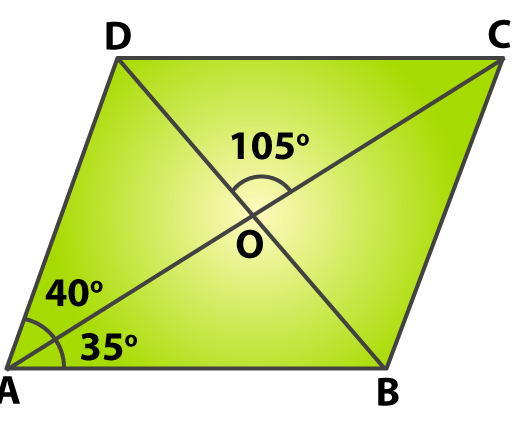Solution:

(i) From the figure we know that ∠ AOB and ∠ COD are vertically opposite angles

So we get

∠ AOB = ∠ COD = 105o

Consider △ AOB

By sum property of a triangle

∠ OAB + ∠ AOB + ∠ ABO = 180o

By substituting the values in above equation

35o + 105o + ∠ ABO = 180o

On further calculation

∠ ABO = 180o – 35o – 105o

By subtraction

∠ ABO = 180o – 140o

∠ ABO = 40o

(ii) We know that AB || DC and BD is a transversal

From the figure we know that ∠ ABD and ∠ CDB are alternate angles

It can be written as

∠ CDO = ∠ CDB = ∠ ABD = ∠ ABO = 40o

So we get

∠ ODC = 40o

(iii) We know that AB || CD and AC is a transversal

From the figure we know that ∠ ACB and ∠ DAC are alternate opposite angles

So we get

∠ ACB = ∠ DAC = 40o

(iv) We know that ∠ B can be written as

∠ B = ∠ CBD + ∠ ABO

So we get

∠ CBD = ∠ B – ∠ ABO

In a parallelogram we know that the sum of all the angles is 360o

So we get

∠ A + ∠ B + ∠ C + ∠ D = 360o

It can be written as

2 ∠ A + 2 ∠ B = 360o

By substituting values in the above equation

2 (40o + 35o) + 2 ∠ B = 360o

On further calculation

2 (75o) + 2 ∠ B = 360o

So we get

150o + 2 ∠ B = 360o

2 ∠ B = 360o – 150o

By subtraction

2 ∠ B = 210o

By division

∠ B = 105o

So we get

∠ CBD = ∠ B – ∠ ABO

By substituting values

∠ CBD = 105o – 40o

By subtraction

∠ CBD = 65o

6. In a parallelogram ABCD, if ∠ A = (2x + 25) o and ∠ B = (3x – 5) o, find the value of x and the measure of each angle of the parallelogram.

Solution:

We know that the opposite angles are equal in a parallelogram

Consider parallelogram ABCD

So we get

∠ A = ∠ C = (2x + 25) o

∠ B = ∠ D = (3x – 5) o

We know that the sum of all the angles of a parallelogram is 360o

So it can be written as

∠ A + ∠ B + ∠ C + ∠ D = 360o

By substituting the values in the above equation

(2x + 25) + (3x – 5) + (2x + 25) + (3x – 5) = 360o

10x + 40o = 360o

By subtraction

10x = 360o – 40o

So we get

10x = 320o

By division we get

x = 32o

Now substituting the value of x

∠ A = ∠ C = (2x + 25) o = (2(32) + 25) o

∠ A = ∠ C = (64 + 25) o

∠ A = ∠ C = 89o

∠ B = ∠ D = (3x – 5) o = (3(32) – 5) o

∠ B = ∠ D = (96 – 5) o

By subtraction

∠ B = ∠ D = 91o

Therefore, x = 32o, ∠ A = ∠ C = 89o and ∠ B = ∠ D = 91o.

7. If an angle of a parallelogram is four fifths of its adjacent angle, find the angles of the parallelogram.

Solution:

Consider ABCD as a parallelogram

If ∠ A = xo

We know that ∠ B is adjacent to A which can be written as 4/5 xo

Opposite angles are equal in a parallelogram

So we get

∠ A = ∠ C = xo and ∠ B = ∠ D = 4/5 xo

We know that the sum of all the angles of a parallelogram is 360o

It can be written as

∠ A + ∠ B + ∠ C + ∠ D = 360o

By substituting the values in the above equation

x + (4/5) x + x + (4/5) x = 360o

2x + (8/5) x = 360o

By taking the LCM as 5

(18/5) x = 360o

By cross multiplication

x = (360 × 5)/18

On further calculation

x = 100o

By substituting the value of x

So we get

∠ A = ∠ C = x = 100o

∠ B = ∠ D = 4/5 xo = (4/5) (100o) = 80o

Therefore, ∠ A = ∠ C = x = 100o and ∠ B = ∠ D = 80o.

8. Find the measure of each angle of a parallelogram, if one of its angles is 30o less than twice the smallest angle.

Solution:

Consider ABCD as a parallelogram

Let us take ∠ A as the smallest angle

So we get

∠ B = 2 ∠ A – 30o

We know that the opposite angles are equal in a parallelogram

∠ A = ∠ C and ∠ B = ∠ D = 2 ∠ A – 30o

We know that the sum of all the angles of a parallelogram is 360o

It can be written as

∠ A + ∠ B + ∠ C + ∠ D = 360o

By substituting the values in the above equation

∠ A + (2 ∠ A – 30o) + ∠ A + (2 ∠ A – 30o) = 360o

On further calculation

∠ A + 2 ∠ A – 30o + ∠ A + 2 ∠ A – 30o = 360o

So we get

6 ∠ A – 60o = 360o

6 ∠ A = 360o + 60o

6 ∠ A = 420o

By division

∠ A = 70o

By substituting the value of ∠ A

∠ A = ∠ C = 70o

∠ B = ∠ D = 2 ∠ A – 30o = 2 (70o) – 30o

∠ B = ∠ D = 110o

Therefore, ∠ A = ∠ C = 70o and ∠ B = ∠ D = 110o.

9. ABCD is a parallelogram in which AB = 9.5 cm and its perimeter is 30 cm. Find the length of each side of the parallelogram.

Solution:

We know that the perimeter of parallelogram ABCD can be written as

Perimeter = AB + BC + CD + DA

We know that opposite sides of parallelogram are equal

AB = CD and BC = DA

By substituting the values

Perimeter = 9.5 + BC + 9.5 + BC

It is given that perimeter = 30 cm

So we get

30 = 19 + 2BC

It can be written as

2BC = 30 – 19

By subtraction

2BC = 11

By division we get

BC = 5.5 cm

Therefore, AB = 9.5 cm, BC = 5.5 cm, CD = 9.5 cm and DA = 5.5 cm.

10. In each of the figures given below, ABCD is a rhombus. Find the value of x and y in each case.

(i)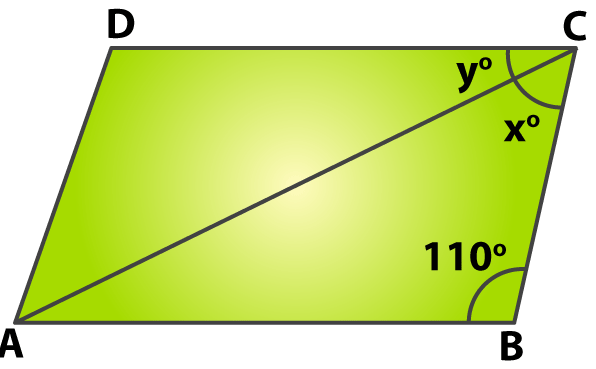(ii)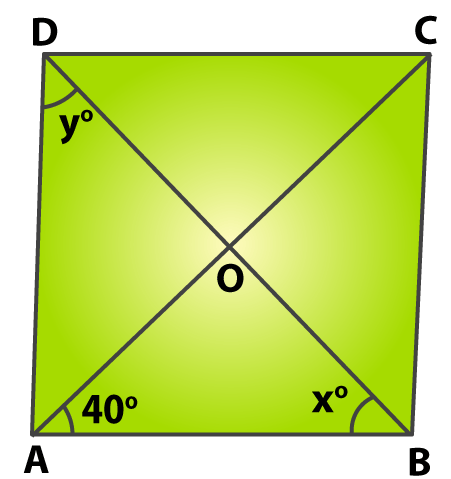(iii)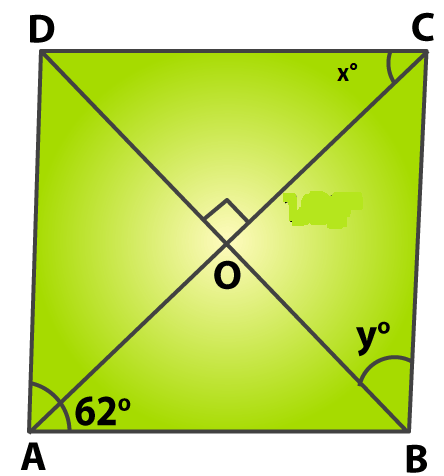Solution:

(i) We know that all the sides are equal in a rhombus

Consider △ ABC

We know that AB = BC

It can be written as

∠ CAB = ∠ ACB = xo

By sum property of a triangle

We get

∠ CAB + ∠ ABC + ∠ ACB = 180o

By substituting the values in above equation

x + 110o + x = 180o

2x + 110o = 180o

On further calculation

2x = 180o – 110o

By subtraction

2x = 70o

By division

x = 35o

Therefore, x = 35o and y = 35o.

(ii) We know that all the sides are equal in a rhombus

Consider △ ABD

We get

It can be written as

x = y ……. (1)

Consider △ ABC

We get

AB = BC and

∠ CAB = ∠ ACB

We know that

∠ ACB = 40o

By using the sum property of a triangle

∠ B + ∠ CAB + ∠ ACB = 180o

By substituting values in the above equation

∠ B + 40o + 40o = 180o

On further calculation

∠ B = 180o – 40o – 40o

By subtraction

∠ B = 180o – 80o

So we get

∠ B = 100o

∠ DBC can be written as

∠ DBC = ∠ B – xo

By substituting the values

∠ DBC = 100o – xo

From the figure we know that ∠ DBC and ∠ ADB are alternate angles

∠ DBC = ∠ ADB = yo

By substituting the value of ∠ DBC

100o – xo = yo

Consider the equation (1) we know that x = y

100o – xo = xo

On further calculation

2xo = 100o

By division

xo = 50o

Therefore, x = y = 50o.

(iii) From the figure we know that

∠ A = ∠ C = 62o

Consider △ BCD

We get

BC = DC

It can be written as

∠ CDB = ∠ DBC = yo

Using the sum property of triangle

∠ BDC + ∠ DBC + ∠ BCD = 180o

By substituting the values

y + y + 62o = 180o

On further calculation

2y = 180o – 62o

By subtraction

2y = 118o

By division

y = 59o

We know that the diagonals of a rhombus are perpendicular to each other

Consider △ COD as a right angle triangle

∠ DOC = 90o

∠ ODC = y = 59o

It can be written as

∠ DCO + ∠ ODC = 90o

To find ∠ DCO

∠ DCO = 90o – ∠ ODC

By substituting the values

∠ DCO = 90o – 59o

∠ DCO = x = 31o

Therefore, x = 31o and y = 59o.

11. The lengths of the diagonals of a rhombus are 24cm and 18 cm respectively. Find the length of each side of the rhombus.

Solution:

We know that ABCD is a rhombus

It is given that AC = 24cm and BD = 18cm

In a rhombus we know that the diagonals bisect each other at right angles

Consider △ AOB

We know that

∠ AOB = 90o

To find AO and BO

We know that

AO = ½ AC

By substituting AC

AO = ½ (24)

AO = 12 cm

BO = ½ BD

By substituting BD

BO = ½ (18)

BO = 9 cm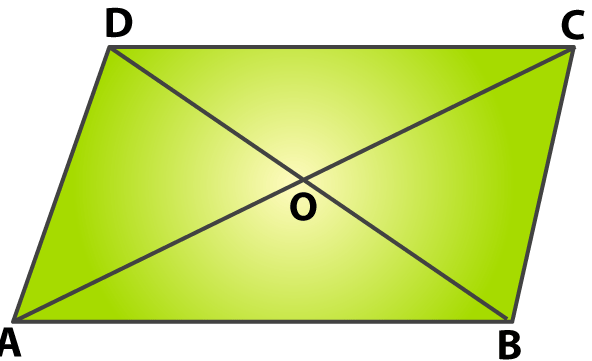Based on the Pythagoras Theorem

AB2 = AO2 + OB2

By substituting values

AB2 = 122 + 92

So we get

AB2 = 144 + 81

AB2 = 225

AB = √ 225

So we get

AB = 15 cm

Therefore, the length of each side of the rhombus is 15 cm.

12. Each side of a rhombus is 10cm long and one of its diagonals measures 16cm. Find the length of the other diagonal and hence find the area of the rhombus.

Solution:

We know that the diagonals of a rhombus bisect at right angles

We get

AO = OC = ½ AC

We know that AC = 16 cm

AO = OC = ½ (16) = 8 cm

Consider △ AOB

Using the Pythagoras Theorem

AB2 = AO2 + OB2

By substituting values

102 = 82 + OB2

On further calculation

OB2 = 100 – 64

By subtraction

OB2 = 36

So we get

OB = √ 36

OB = 6 cm

We know that the length of other diagonal

BD = 2 × OB

By substituting the value

BD = 2 × 6

BD = 12 cm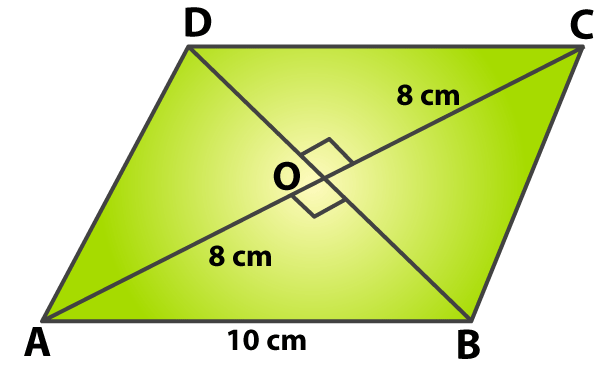From the figure the area of

△ ABC = ½ × AC × OB

By substituting the values

△ ABC = ½ × 16 × 6

By multiplication

△ ABC = 48 cm2

△ ACD = ½ × AC × OD

By substituting the values

△ ACD = ½ × 16 × 6

By multiplication

△ ACD = 48 cm2

So we can calculate the area of rhombus as

Area of rhombus = area of △ ABC + area of △ ACD

We get

Area of rhombus = 48 + 48 = 96 cm2

Therefore, the length of other diagonal is 12 cm and the area of rhombus is 96 cm2.

13. In each of the figures given below, ABCD is a rectangle. Find the values of x and y in each case.

(i)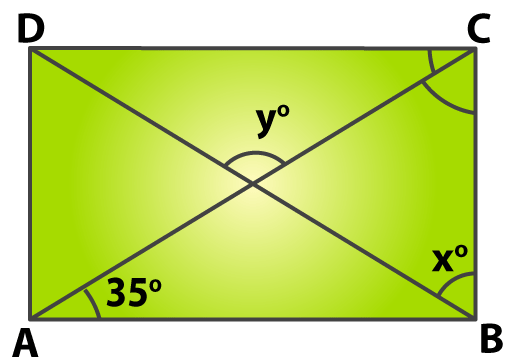(ii)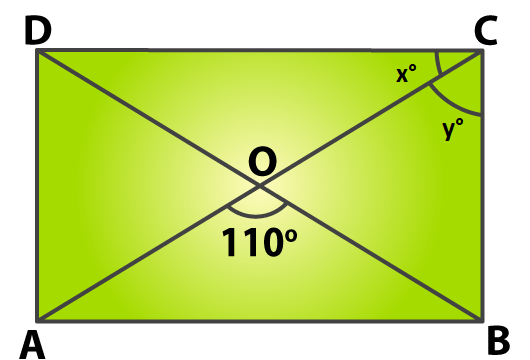Solution:

(i) From the figure we know that the diagonals are equal and bisect at point O.

Consider △ AOB

We get AO = OB

We know that the base angles are equal

∠ OAB = ∠ OBA = 35o

By using the sum property of triangle

∠ AOB + ∠ OAB + ∠ OBA = 180o

By substituting the values we get

∠ AOB + 35o + 35o = 180o

On further calculation

∠ AOB = 180o – 35o – 35o

By subtraction

∠ AOB = 180o – 70o

∠ AOB = 110o

From the figure we know that the vertically opposite angles are equal

∠ DOC = ∠ AOB = y = 110o

In △ ABC

We know that ∠ ABC = 90o

Consider △ OBC

We know that

∠ OBC = xo = ∠ ABC – ∠ OBA

By substituting the values

∠ OBC = 90o – 35o

By subtraction

∠ OBC = 55o

Therefore, x = 55o and y = 110o.

(ii) From the figure we know that the diagonals of a rectangle are equal and bisect each other.

Consider △ AOB

We get

OA = OB

We know that the base angles are equal

∠ OAB = ∠ OBA

By using the sum property of triangle

∠ AOB + ∠ OAB + ∠ OBA = 180o

By substituting the values

110o + ∠ OAB + ∠ OBA = 180o

We know that ∠ OAB = ∠ OBA

So we get

2 ∠ OAB = 180o – 110o

By subtraction

2 ∠ OAB = 70o

By division

∠ OAB = 35o

We know that AB || CD and AC is a transversal

From the figure we know that ∠ DCA and ∠ CAB are alternate angles

∠ DCA = ∠ CAB = yo = 35o

Consider △ ABC

We know that

∠ ACB + ∠ CAB = 90o

So we get

∠ ACB = 90o – ∠ CAB

By substituting the values in above equation

∠ ACB = 90o – 35o

By subtraction

∠ ACB = x = 55o

Therefore, x = 55o and y = 35o.

14. In a rhombus ABCD, the altitude from D to the side B bisects AB. Find the angles of the rhombus.

Solution: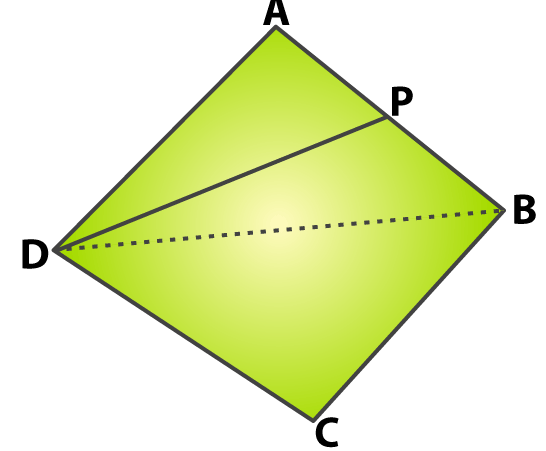From the figure consider DP bisects the side AB at point P

Construct a line at BD

Consider △ AMD and △ BMD

We know that M is the mid-point of AB

AM = BM

From the figure we know that

∠ AMD = ∠ BMD = 90o

MD is common i.e. MD = MD

By SAS congruence criterion

△ AMD ≅ △ BMD

AD = BD (c. p. c. t)

We know that the sides of a rhombus are equal

So we get

It can be written as

We know that △ ADB is an equilateral triangle

So we get

∠ A = 60o

From the figure we know that the opposite angles are equal

∠ C = ∠ A = 60o

We know that

∠ B + ∠ A = 180o

On further calculation

∠ B = 180o – ∠ A

∠ B = 180o – 60o

By subtraction

∠ B = 120o

We know that ∠ D = ∠ B = 120o

Therefore, the angles are ∠ C = ∠ A = 60o and ∠ D = ∠ B = 120o.

15. In the adjoining figure, ABCD is a square. A line segment CX cuts AB at X and the diagonal BD at O such that ∠ COD = 80o and ∠ OXA = xo. Find the value of x.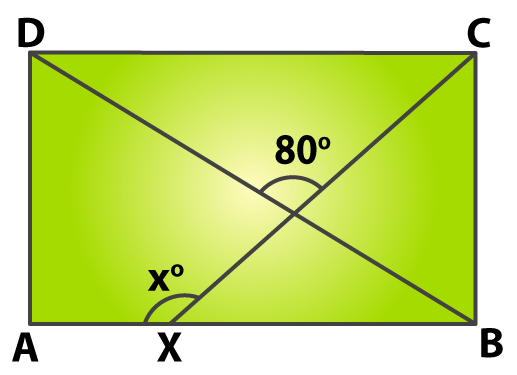Solution:

In △ ABD

We know that AB = AD

From the figure we know that the base angles are equal

We know that ∠ A = 90o

It can be written as

∠ ADB + ∠ ABD = 90o

We know that ∠ ADB = ∠ ABD

So we get

By division

Consider △ OXB

From the figure we know that ∠ XOB and ∠ DOC are vertically opposite angles

∠ XOB = ∠ DOC = 80o

We also know that

∠ ABD = ∠ XBD = 45o

We can write it as

Exterior ∠ AXO = ∠ XOB + ∠ XBD

By substituting the values

xo = 80o + 45o

xo = 125o

Therefore, the value of x is 125o.

16. In a rhombus ABCD show that diagonal AC bisects ∠ A as well as ∠ C and diagonal BD bisects ∠ B as well as ∠ D.

Solution: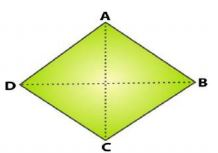Consider △ ABC and △ ADC

We know that the sides of rhombus are equal

AB = AD and BC = CD

AC is common i.e. AC = AC

By SSS congruence criterion

We know that

∠ BAC = ∠ DAC and ∠ BCA = ∠ DCA (c. p. c. t)

Therefore, AC bisects ∠ A as well as ∠ C.

Consider △ BAD and △ BCD

We know that the sides of rhombus are equal

AB = BC and AD = CD

BD is common i.e. BD = BD

By SSS congruence criterion

We know that

∠ ABD = ∠ CBD and ∠ ADB = ∠ CDB (c. p. c. t)

Therefore, BD bisects ∠ B as well as ∠ D.

17. In a parallelogram ABCD, points M and N have been taken on opposite sides AB and CD respectively such that AM = CN. Show that AC and MN bisect each other.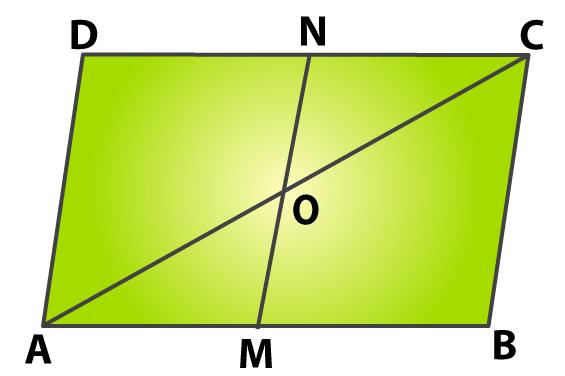Solution:

Consider △ AMO and △ CNO

We know that AB || CD

From the figure we know that ∠ MAO and ∠ NCO are alternate angles

It is given that AM = CN

We know that ∠ AOM and ∠ CON are vertically opposite angles

∠ AOM = ∠ CON

By ASA congruence criterion

△ AMO ≅ △ CNO

So we get

AO = CO and MO = NO (c. p. c. t)

Therefore, it is proved that AC and MN bisect each other.

18. In the adjoining figure, ABCD is a parallelogram. If P and Q are points on AD and BC respectively such that AP = 1/3 AD and CQ = 1/3 BC, prove that AQCP is a parallelogram.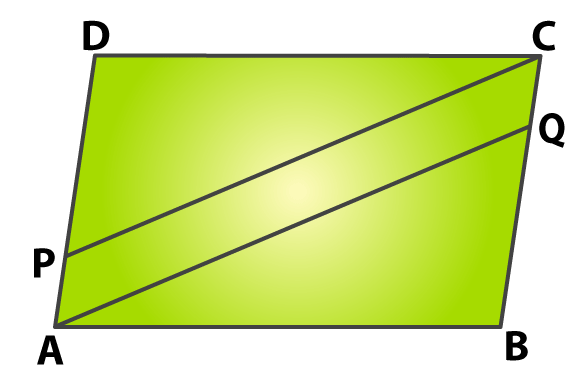Solution:

Consider △ ABQ and △ CDP

We know that the opposite sides of a parallelogram are equal

AB = CD

So we get ∠ B = ∠ D

We know that

BQ = BC – CQ

i.e. BQ = BC – 1/3 BC

BQ = (3-1)/3 BC

We know that AD = BC

So we get

BQ = 2/3 BC = 2/3 AD

We get BQ = DP

By SAS congruence criterion

△ ABQ ≅ △ CDP

AQ = CP (c. p. c. t)

We know that

We know that AD = BC

CQ = 1/3 BC = 1/3 AD

So we get

PA = CQ

∠ QAB = ∠ PCD (c. p. c. t)… (1)

We know that

∠ QAP = ∠ A – ∠ QAB

Consider equation (1)

∠ A = ∠ C

∠ QAP = ∠ C – ∠ PCD

From the figure we know that the alternate interior angles are equal

∠ QAP = ∠ PCQ

So we know that AQ and CP are two parallel lines.

Therefore, it is proved that PAQC is a parallelogram.

19. In the adjoining figure, ABCD is a parallelogram whose diagonals intersect each other at O. A line segment EOF is drawn to meet AB at E and DC at F. Prove that OE = OF.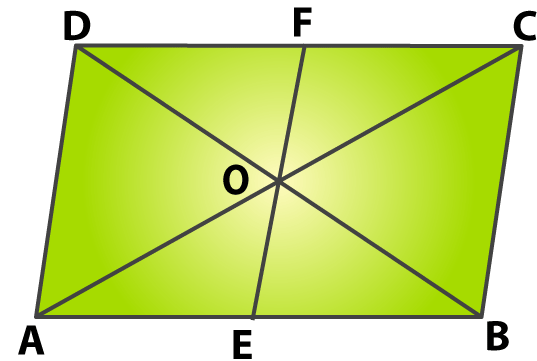Solution:

We know that ABCD is a parallelogram whose diagonals intersect each other at O

Consider △ AOE and △ COF

We know that ∠ CAE and ∠ DCA are alternate angles

From the figure we know that the diagonals are equal and bisect each other

AO = CO

We know that ∠ AOE and ∠ COF are vertically opposite angles

∠ AOE =∠ COF

By ASA congruence criterion

△ AOE ≅ △ COF

OE = OF (c. p. c. t)

Therefore, it is proved that OE = OF.

20. The angle between two altitudes of a parallelogram through the vertex of an obtuse angle of the parallelogram is 60o. Find the angles of the parallelogram.

Solution: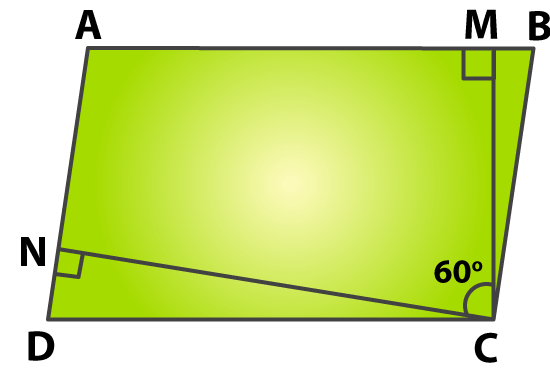From the figure

∠ DCM = ∠ DCN + ∠ MCN

By substituting values in the above equation

90o = ∠ DCN + 60o

On further calculation

∠ DCN = 90o – 60o

By subtraction

∠ DCN = 30o

Consider △ DCN

Using the sum property

∠ DNC + ∠ DCN + ∠ D = 180o

By substituting values in the above equation

90o + 30o + ∠ D = 180o

On further calculation

∠ D = 180o – 90o – 30o

By subtraction

∠ D = 180o – 120o

∠ D = 60o

We know that the opposite angles of parallelogram are equal

∠ B = ∠ D = 60o

It can be written as

∠ A + ∠ B = 180o

By substituting values

∠ A + 60o = 180o

By subtraction

∠ A = 180o – 60o

∠ A =120o

So we get ∠ A = ∠ C =120o

Therefore, ∠ B = ∠ D = 60o and ∠ A = ∠ C =120o.

21. ABCD is a rectangle in which diagonal AC bisects ∠ A as well as ∠ C. Show that

(i) ABCD is a square,

(ii) Diagonal BD bisects ∠ B as well as ∠ D.

Solution: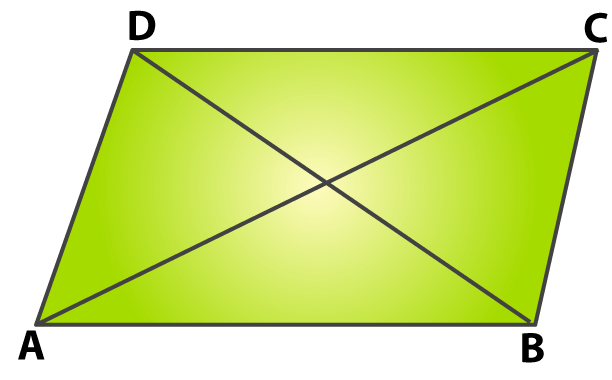(i) We know that ABCD is a rectangle in which diagonal AC bisects ∠ A as well as ∠ C.

So we get

∠ BAC = ∠ DAC …….. (1)

∠ BCA = ∠ DCA …….. (2)

We know that every rectangle is a parallelogram

So we get AB || DC and AC is a transversal

From the figure we know that ∠ BAC and ∠ DCA are alternate angles

∠ BAC = ∠ DCA

By considering equation (1)

We get

∠ DAC = ∠ DCA

We know that the opposite sides of equal angles are equal

Since ABCD is a rectangle

We get AB = BC and CD = AD

So we get AB = BC = CD = AD

Therefore, it is proved that ABCD is a square.

(ii) Consider △ BAD and △ BCD

We know that AB = CD and AD = BC

BD is common i.e. BD = BD

By SSS congruence criterion

We know that

∠ ABD = ∠ CBD and ∠ ADB = ∠ CDB (c. p. c. t)

Therefore, diagonal BD bisects ∠ B as well as ∠ D.

22. In the adjoining figure, ABCD is a parallelogram in which AB is produced to E so that BE = AB. Prove that ED bisects BC.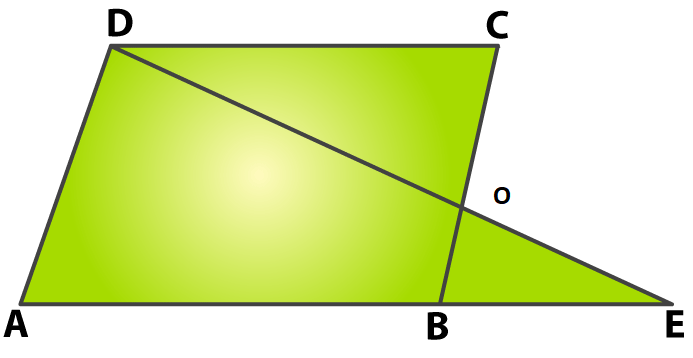Solution:

Consider △ OCD and △ OBE

From the figure we know that ∠ DOC and ∠ EOB are vertically opposite angles

∠ DOC = ∠ EOB

We know that AB || CD and BC is a transversal

∠ OCD and ∠ OBE are alternate angles

∠ OCD = ∠ OBE

From the figure we know that AB = CD and BE = AB

So we can write DC = BE

By AAS congruence criterion

△ OCD ≅ △ OBE

OC = OB (c. p. c. t)

Therefore, it is proved that ED bisects BC.

23. In the adjoining figure, ABCD is a parallelogram and E is the midpoint of side BC. If DE and AB when produced to meet at F, prove that AF = 2AB.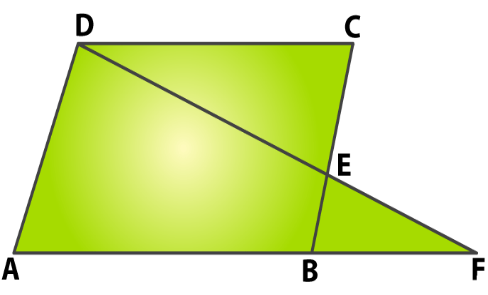Solution:

Consider △ DEC and △ FEB

From the figure we know that ∠ DEC and ∠ FEB are vertically opposite angles

∠ DEC = ∠ FEB

∠ DCE and ∠ FBE are alternate angles

∠ DCE = ∠ FBE

It is given that CE = EB

By AAS congruence criterion

△ DEC ≅ △ FEB

DC = FB (c. p. c. t)

From the figure

AF = AB + BF

We know that BF = DC and AB = DC

So we get

AF = AB + DC

AF = AB + AB

AF = 2AB

Therefore, it is proved that AF = 2AB.

24. Two parallel lines l and m are intersected by a transversal t. Show that the quadrilateral formed by the bisectors of interior angles is a rectangle.

Solution: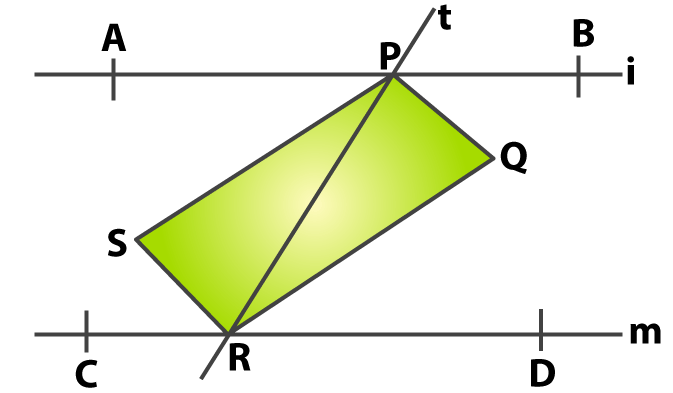We know that l || m and t is a transversal

From the figure we know that ∠ APR and ∠ PRD are alternate angles

∠ APR = ∠ PRD

We can write it as

½ ∠ APR = ½ ∠ PRD

We know that PS and RQ are the bisectors of ∠ APR and ∠ PRD

So we get

∠ SPR = ∠ PRQ

Hence, PR intersects PS and RQ at points P and R respectively

We get

PS || RQ

In the same way SR || PQ

Therefore, PQRS is a parallelogram

We know that the interior angles are supplementary

∠ BPR + ∠ PRD = 180o

From the figure we know that PQ and RQ are the bisectors of ∠ BPR and ∠ PRD

We can write it as

2 ∠ QPR + 2 ∠ QRP = 180o

Dividing the equation by 2

∠ QPR + ∠ QRP = 90o …… (1)

Consider △ PQR

Using the sum property of triangle

∠ PQR + ∠ QPR + ∠ QRP = 180o

By substituting equation (1)

∠ PQR + 90o = 180o

On further calculation

∠ PQR = 180o – 90o

By subtraction

∠ PQR = 90o

We know that PQRS is a parallelogram

It can be written as

∠ PQR = ∠ PSR = 90o

We know that the adjacent angles in a parallelogram are supplementary

∠ SPQ + ∠ PQR = 180o

By substituting the values in above equation

∠ SPQ + 90o = 180o

On further calculation

∠ SPQ = 180o – 90o

By subtraction

∠ SPQ = 90o

We know that all the interior angles of quadrilateral PQRS are right angles

Therefore, it is proved that the quadrilateral formed by the bisectors of interior angles is a rectangle.

25. K, L, M and N are points on the sides AB, BC, CD and DA respectively of a square ABCD such that AK = BL = CM = DN. Prove that KLMN is a square.

Solution: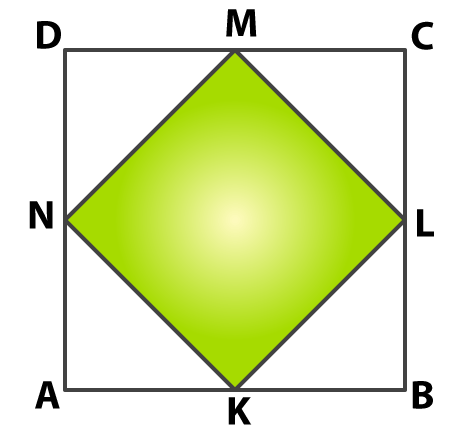It is given that AK = BL = CM = DN

ABCD is a square

So we get

BK = CL = DM = AN …… (1)

Consider △ AKN and △ BLK

It is given AK = BL

From the figure we know that ∠ A = ∠ B = 90o

Using equation (1)

AN = BK

By SAS congruence criterion

△ AKN ≅ △ BLK

We get

∠ AKN = ∠ BLK and ∠ ANK = ∠ BKL (c. p. c. t)

We know that

∠ AKN + ∠ ANK = 90o

∠ BLK + ∠ BKL = 90o

∠ AKN + ∠ ANK + ∠ BLK + ∠ BKL = 90o + 90o

On further calculation

2 ∠ ANK + 2 ∠ BLK = 180o

Dividing the equation by 2

∠ ANK + ∠ BLK = 90o

So we get

∠ NKL = 90o

In the same way

∠ KLM = ∠ LMN = ∠ MNK = 90o

Therefore, it is proved that KLMN is a square.

26. A △ ABC is given. If lines are drawn through A, B, C, parallel respectively to the sides BC, CA and AB, forming △ PQR, as shown in the adjoining figure, show that BC = ½ QR.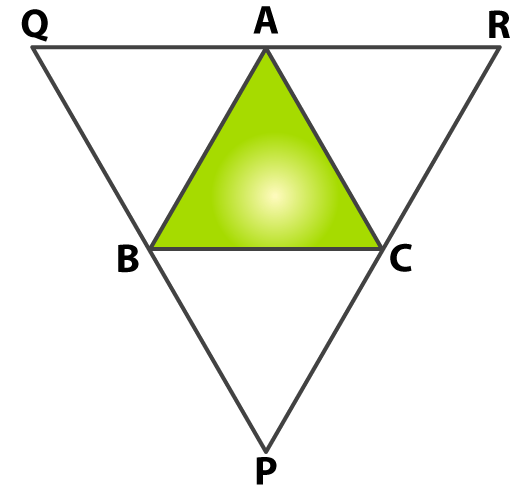Solution:

We know that AR || BC and AB || RC

From the figure we know that ABCR is a parallelogram

So we get

AR = BC …… (1)

We know that AQ || BC and QB || AC

From the figure we know that AQBC is a parallelogram

So we get

QA = BC ……… (2)

AR + QA = BC + BC

We know that AR + QA = QR

So we get

QR = 2BC

Dividing by 2

BC = QR/2

BC = ½ QR

Therefore, it is proved that BC = ½ QR.

27. In the adjoining figure, △ ABC is a triangle and through A, B, C lines are drawn, parallel respectively to BC, CA and AB, intersecting at P, Q and R. Prove that the perimeter of △ PQR is double the perimeter of △ ABC.Solution:

We know that AR || BC and AB || RC

From the figure we know that ABCR is a parallelogram

So we get

AR = BC …….. (1)

We know that AQ || BC and QB || AC

From the figure we know that AQBC is a parallelogram

So we get

QA = BC ……… (2)

By adding both the equations we get

AR + QA = BC + BC

We know that AR + QA = QR

So we get

QR = 2BC

It can be written as

BC = QR/2

BC = ½ QR

In the same way

AB = ½ RP and AC = ½ PQ

Perimeter of △ PQR = PQ + QR + RP

It can be written as

Perimeter of △ PQR = 2AC + 2BC + 2AB

By taking 2 as common

Perimeter of △ PQR = 2 (AC + BC + 2AB)

Perimeter of △ PQR = 2 (Perimeter of △ ABC)

Therefore, it is proved that the perimeter of △ PQR is double the perimeter of △ ABC.

### Access other exercise solutions of Class 9 Maths Chapter 10: Quadrilaterals

Exercise 10A Solutions 10 Questions

Exercise 10C Solutions 18 Questions

### RS Aggarwal Solutions Class 9 Maths Chapter 10 – Quadrilaterals Exercise 10B

RS Aggarwal Solutions Class 9 Maths Chapter 10 Quadrilaterals Exercise 10B consists of theorems which are based on various structures like parallelogram, rhombus, rectangle and square.

### Key features of RS Aggarwal Solutions for Class 9 Maths Chapter 10: Quadrilaterals Exercise 10B

• The solutions are prepared in simple language based on the understanding capacity of students.
• By referring the PDF, students can improve their logical thinking abilities, which is important in solving problems.
• RS Aggarwal solutions are well designed by the teachers with various methods used for a single problem.
• They mainly help students to complete the entire syllabus and prepare effectively for their board exams.